As of today, I will have spent precisely half of my life at $PLACE_OF_WORK. I first arrived at what would become my workplace as a badly coiffured youth in 1995 to do a biology degree. South Kensington seemed a great improvement over Croydon, where I had endured my previous 18 years: there was a refreshing absence of casual street violence, and a greatly improved proximity to the grubby delights of Soho. At that time, my Hall of Residence was directly above the first-year lecture theatre, and in the same building as the Students’ Union. Despite this tempting proximity to cheap vodka – and even cheaper dates – I somehow managed to attend almost every lecture of my first year, aside from a week (a week!) of lectures on algae, which I traded for bossing Munchkins about in the Questor’s Theatre in Ealing. I met my personal tutor at least twice, survived two under-catered field-trips to somewhere, somewhere in a field in HampshireBerkshire, and made friends whom I treasure to this day.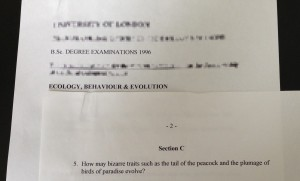I discovered a cache of mediaeval exam papers in the bottom of a filing cabinet when I last cleared out my office. I have a very distinct memory of answering this question, probably because it involved talking about the “Sexy Sons” hypothesis. Second-year forced me out into less convenient accommodation: an ill-conceived double-Georgian knock-through near Brompton Cemetery with 18 bedrooms, and anything up to 2 working bathrooms on any given day. Due to sometwo else both failing their first-year exams, I found myself promoted to Homosexual in Chief of the LGBT society, for which dubious honour I now have a pot behind the Union bar.I am number 2 on the list of Chief Homosexuals. I believe number 1 is now advising the Lib Dems on election strategy. I suspect this pot may be cursed. My final-year project on copper-tolerant fungi somewhere, somewhere in that field in Berkshire led to the offer of a PhD in wood preservation, which I leapt upon, having received no careers guidance whatsoever up to that point, and having begun to fear moving back to Croydon for want of any botanical PhD opportunities in London. My undergraduateship ended with a viva voce, upon which I thought hung the fate of my entire degree; in fact, I turned out to be a control, and what I had thought would be a bowel-loosening grilling turned out to be entirely unmemorable.I had to hire my suit for the Summer Ball just before I graduated. I still hate wearing suits of any kind, which probably contributes to my unemployability outside of academia. Like most postgraduate research degrees, mine was a heady mix of disappointment, poverty, and the growing realisation that week-day nights-out were incompatible with competent laboratory work. My department had moved out of the timeshare flat with the Students’ Union and into a brand-new building during the summer between my BSc and PhD, but someone had been a little unrealistic about the space available in the new labs. The first and second years of my PhD were spent trying not to poison myself with arsenic trioxide amongst a labyrinth of broken vacuum impregnators, quickfit glassware, and bottles of solvent with labels written in Linear A; the third and fourth years spent trying to fit research into the gaps between the demonstrating in lab practicals I had to do in order to have enough money to eat. Somehow I captured the heart of a young aeronautical engineer, who has miraculously put up with my questionable charms ever since. I presented my ground-breaking findings on the bacterial biotransformation of an anti-sapstain chemical to a conference in glamorous Cardiff, and left it at that. My contribution to the greater knowledge of humankind will forever be a few grey literature conference proceedings, and a large blue book buried in quicklime below the College library.I occasionally have nightmares about being buried under a landslide of poorly preserved pallet boards. Having drifted into a PhD, I continued on my under-thought career path by applying for a three-year post-doctoral position that combined part-time research with a part-time PGCE in secondary school education. In retrospect, combining the laugh-a-minute relaxation of academic research with the delights of herding teenagers through GCSEs may not have been the best life decision I’ve made. There were amusing moments – the attempts of year 7 students to embarrass me during sex-ed lessons were doomed from the start – but mostly it was exhausting and impossible. I somehow made it through to the other side, but with no interest whatsoever in ever darkening the door of a secondary school or research lab again.Simulating woodlice: anything was better than differentiating my citizenship lessons for kinaesthetic learners [sic] Fortunately, I had kept up a bit of lab demonstrating on the side, and had even been roped into giving a few first-year lectures in the twilight of my PhD. A temporary position opened up convening a first-year biology course, giving a few lectures, and running some of the practicals I’d been demonstrating for the best part of a decade. And so began a slow accretion from ‘stop-gap teaching gimp’ to ‘senior teaching fellow’. Many of the staff who taught me as an undergrad have since retired or moved on; even the new-born building of 1998 is now old enough to legally have sex and drive a moped. Some 1700 students have learned – or at least endured – first-year molecular biology and enzymology with me, and the pile of marking in front of me (for which writing this banal drivel is the sort of displacement activity against which I’ve hypocritically warned those very students) probably contains the ten thousandth script I have scrawled with the Biros of judgement. I probably ought to get back to it. In confirmation of the universe’s pitiless malevolence, I now give the lectures on algae that I skived off in my first-year.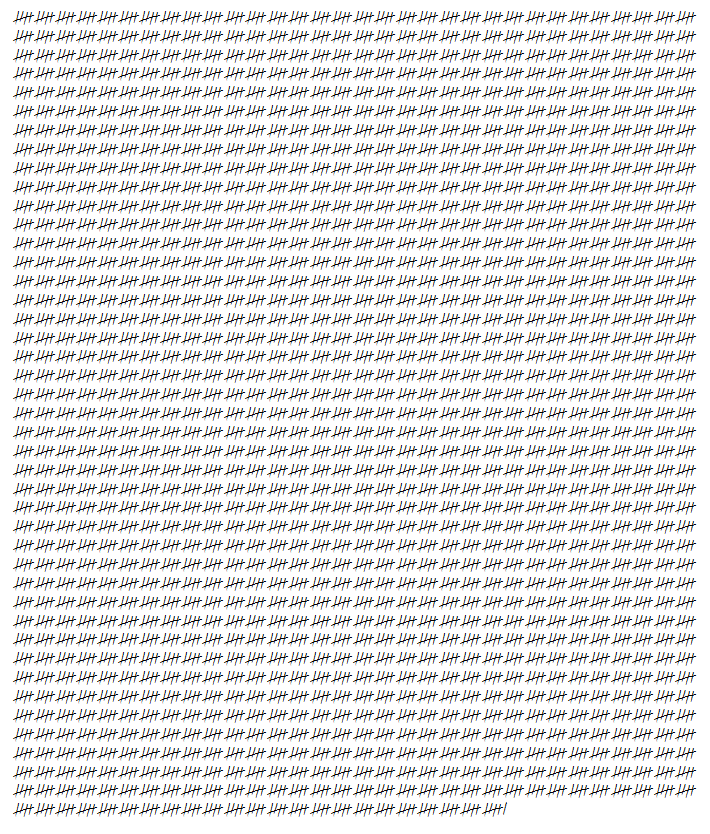Aleph-naught bottles of beer on the wall, aleph-naught bottles of beer, you take one down, you hand it on round, aleph-naught bottles of beer on the wall ## Organism of the week #21 – Flying machines It is frequently, and largely accurately, said that an area of Amazon rainforest the size of Wales is deforested every year. Horrendous though this statistic is, it’s worth remembering that the UK deforested an area at least the size of Wales (including most of the area commonly known as “Wales”) before anyone started keeping notes. The UK’s track record at maintaining its biodiversity has been – to put it generously – somewhat patchy. We have wiped out a goodly swathe of our large mammals: brown bears, elks, lynxes, and wolves; we drove our blue backed stag beetles to oblivion; and Davall’s Sedge has not been spotted since 1930. One species that was formerly so common in the UK that Shakespeare felt the need to warn theatre-goers about its favoured nest-building materials is the red kite: My Trafficke is sheetes: when the Kite builds, looke to lesser Linnen. This beautiful bird was very nearly wiped out in the UK by the early 20th century; only a handful of breeding pairs were left by 1990, in – you guessed it – Wales. Its populations in southern Europe continue to decline, and it is still considered near threatened. However, since the 1990s, the red kite has been the target of a major reintroduction program in the UK, and in a few places they are once again a common sight, soaring on thermals and seeking out rabbits, carrion, and recently washed pillowcases. A good place to see these impressive birds is the Chilterns, a range of chalk hills just north of London. I’m not generally a charismatic-megafauna kind of biologist, but getting close enough for even this somewhat blurry action shot was thrilling:Naturally-selected flying machine: red kite (Milvus milvus), unimpressed by the launch of a glider over the top of its head The kites particularly like to hang around on airfields, presumably on the look-out for tasty pilots. Their blasé attitude to the planes and gliders is amusing if you’re on the ground. It is somewhat less amusing when you meet them in the air, and they remind you in no uncertain terms that their lineage has been flying since before your lineage even took to the trees, let alone came back down from them.Human-designed flying machines. Well, one’s a glider, which is to flying what a snake is to tap-dancing, but you know what I mean. ## Bagging botanic gardens I’m not sure whether bagging botanical gardens is better or worse than bagging Munros, Michelin stars or the numbers off of rolling stock, but it keeps me off the streets… # London (Kew) Just ten stops down the District Line from$WORK lies the Royal Botanic Gardens Kew. The gardens have three enormous glasshouses, a number of smaller glasshouses, and 121 hectares of trees, beds and desperately awful architecture to explore. Unfortunately, it also has an entry fee (for non-concession adults) of £14.50, which is a little steep, and possibly one of the reasons that disappointingly few of my students seem to have visited it, despite its proximity.

My favourite indoor displays at Kew are the two rooms of carnivorous plants in the Princess of Wales Conservatory (don’t miss the newer cloud-forest full of Nepenthes), and the ever-changing contents of the Alpine and Waterlily Houses. The latter often has large clumps of sensitive plants (Mimosa pudica and relatives) to poke. I also enjoy the very Victorian approach to health-and-safety in the walkway at the top of the Palm House.

Botanerd highlights.

1. Play “palm or cycad?” at the two ends of the Palm House.
2. Flowers are essentially tarts. Prostitutes for the bees. The Princess of Wales Conservatory has a very good selection of huge flower-free ferns and spikemosses (Selaginella).
3. There’s a gigantic Ginkgo just round the back of the Princess of Wales Conservatory; and the oft ignored (and therefore much less busy) far end of the gardens has a fantastic collection of conifers, including AraucariaSequoia, Sequoiadendron, Torreya, Cunninghamia, and Cryptomeria, in addition to all the yews, cypresses, pines, firs, larches, cedars and spruces you can eat.

London (Chelsea)

Small but perfectly formed, the Chelsea Physic Garden is one of the oldest botanic gardens in the world (Oxford, below, claims the top spot). It specialises in plants used by humans, including (when I last went) a special display of plant fibre ropes. Entry about £10.Hemp rope smells nicest and can be drawn very fast over skin without causing friction burns, which is an important consideration for, erm, rigging – yes – rigging

Botanerd highlights.

1. Count the number of exciting ways you could get killed by the plants in the Pharmaceutical Garden display.

# Edinburgh

I don’t remember the Royal Botanic Garden Edinburgh being this sunny either time we visited, but apparently it was on at least one trip. Unlike Kew, the glasshouses are squidged together, so if the weather’s misbehaving, your fern to rain ratio will be much higher than in London. Unfortunately, like Kew, at the time of writing, some of the glasshouses are shut for renovations. Console yourself with the fact that entry to the gardens themselves is free.

Botanerd highlights.

1. Edinburgh is the only place I’ve ever seen a clubmoss (Lycopodium) on display. See if it’s still there.
2. It’s also the only place I’ve ever seen a Gnetum (picture at the end of this post).

# Barcelona

Sitting on the slopes of Montjuïc, just below the Olympic Stadium, the Jardí Botànic de Barcelona is my most recent bagging. Unlike the other gardens here, it is entirely outdoors, with no glasshouses, and therefore specialises in plants from Mediterranean scrub habitats like Chile, South West Australia and California. Entry fee to the gardens is a very reasonable €3.

Botanerd highlights.

1. Australian grass-trees and giant Chilean Puya bromeliads.
2. As you’re on the side of a hill, the views are also fantastic, and the easiest way to get there is via cable-car, so you get to soar over the local conifers too.

# Amsterdam

The photo below of De Hortus Botanicus Amsterdam doesn’t do it justice, but it’s well worth the €8.50 entry. The glasshouses are very well laid out, and they have a very good selection of carnivorous plants, obscure ferns (including Marattia) and cycads.

Botanerd highlights.

1. The aforementioned carnivorous plants and obscure ferns.
2. I wonder what this could possibly be?

# Oxford

Claiming to be the oldest botanic garden in the world (and I’ve no reason to doubt them!), the University of Oxford Botanic Garden is a snip at £4.50 entry, and has a good mixture of outdoor beds and glasshouses. The glasshouses are small, but absolutely rammed with stuff, including Pachypodium (below), assorted ferns, jade vines, a lovely Amorphophallus rivieri (well, lovely until you stick your nose over it), but – as it turns out – no Orchis fatalis.

Botanerd highlights.

1. This is the only place I’ve ever seen a Psilotum, which had me squealing with excitement, much to the disdain and bafflement of my long-suffering companion on these trips.
2. Like the rest of this garden, the carnivorous plant glasshouse crams a lot of variety into a small space.

# Berlin

The Botanischer Garten und Botanisches Museum Berlin-Dahlem claims to be the second-largest in the world (after Kew), and now has a dedicated moss garden (which unfortunately post-dates my visit) as well as the usual beds and (extensive) glasshouses. Entry fee is €6. UPDATE – I have now seen the moss garden with my own eyes and it makes me weep with happiness.

Botanerd highlights.

1. Several of the botanic gardens mentioned above cultivate Welwitschia mirabilis, a very strange plant from the Namib that grows only two enormous strap-like leaves in its lifetime, but only Berlin seems to have been completely successful: their plants are verdant and frequently in flower (‘in cone’, really, as this plant is closely related to the pines and other conifers).

# (Dis)honourable mentions

I didn’t quite make it into the San Francisco Botanical Garden, but perhaps one day I’ll return with more time, and having not been recently fleeced at the California Academy of Sciences ($30 entry!) Brussels has a wholly confusing pair of botanic gardens, the National Botanic Garden of Belgium, which is just north of Brussels, and the Botanical Garden of Brussels, which sits on the real botanic garden’s old site in the middle of Brussels. I got the former mixed up with the latter, much to my disappointment. It’s perfectly pleasant, but not really a botanic garden. Darwin’s House at Down in Kent has a small glasshouse with a good collection of carnivorous plants. Well worth a visit, and a wander down the sandwalk. # Where next? In particular, I’d love to know where I can see the following obscure corners of the vegetable empire: • Ophioglossum or Botrychium (adders-tongue fern). • Hornworts (Anthoceros), and/or a really good moss and liverwort display (preferably closer than Berlin!) • Quillworts (Isoetes). • Amborella. UPDATE – spotted at Berlin! • Utricularia tenella or Utricularia multifida (previously Polypompholyx tenella and Polypompholyx multifida, until Peter Taylor cast the fairy aprons into the eternal darkness of taxonomic obsolescence). ## Organism of the week #20 – Don’t point that thing at me It’s amazing how informative an anus can be. Take this sea urchin. The orange pucker in the middle of the spines is its “around-the-bum”, although zoologists would insist on writing that in Greek as “periproct“. The bright orange ring-piece is characteristic of this species, and marks it out as Diadema setosum, rather than any of the less rectally blessed species of Diadema. The butt-hole of an urchin is actually the second it will own, because urchins go through a metamorphosis that shames even that of a butterfly. The larva of an urchin looks not even a little bit like the adult…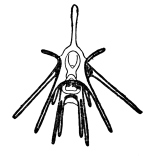Pluteus larva of a sea urchin. The adult will develop as a ball inside the larva’s body. The spikes on the larva are nothing to do with the spikes on the adult …and the adult urchin develops like a well-organised tumour within the body of the larva. For this reason, the adult’s anus is an entirely different hole from the larva’s anus. The development of the larva’s original butt-hole during development from a fertilised egg turns out to be quite revealing. Surprisingly, it marks out sea urchins and their relatives – like sea cucumbers and starfish – as much closer relatives of yours and other backboned animals, than they are of insects or worms or jellyfish, or indeed, or pretty much any other animal. As a fertilised human or sea urchin egg divides, it forms a hollow ball of cells, somewhat like a football. Then, some of the cells on the surface fold in on themselves, forming a shape rather like what you get if you punch your fist into a half-deflated football. The dent drills its way through, and eventually opens out through the other side of the ball. What you end up with is a double-walled tube, with a hole at either end.In humans and all other animals with backbones, and in the larva of sea urchins and starfish and sea cucumbers, the first hole – the one formed by the dent – becomes the anus; and the second hole – where the dent punches through to the other side – becomes the mouth. In most other animals, the first hole becomes the mouth, and the second the anus (pedant alert: I’m glossing over some details here). Humans and sea urchins develop arse-first. Or mouth-second, as zoologists would prudishly have it, preferably euphemised further by writing it in Greek. Humans and fish, and sea urchins and starfish are all “deuterostomes”. The development of the chocolate starfish of a starfish and of the asshole of an ass hint at a deep evolutionary connection between two very different groups of animals. Enlightenment can be found in the most unexpected places. ## Nonlinear regression Nonlinear regression is used to see whether one continuous variable is correlated with another continuous variable, but in a nonlinear way, i.e. when a set of x vs. y data you plan to collect do not form a straight line, but do fall on a curve that can be modelled in some sensible way by a known equation, e.g.$v = \frac{ v_{max} \cdot [S] }{ K_M + [S] }$ Some important general considerations for fitting models of this sort include: • The model must make physical sense. R (and Excel) can happily stick polynomial curves (e.g. a cubic like y=ax3+bx2+cx+d) through a data set, but fitting random equations through data is a pointless exercise, as the values of a, b, c and d are meaningless and do not relate to some useful quantity that characterises the behaviour of the data. If you want to fit a curve to a data set, it has to be a curve (and therefore an equation) you’ve chosen because it estimates something meaningful (e.g. the Michaelis constant, KM). • There must be enough data points. In general, you cannot fit a useful model of n parameters to a data set smaller than n+1 in size. In linear regression, you cannot fit a slope and an intercept (2 parameters) to just one datum, as there are an infinite number of lines that pass through a single point and no way to choose between them. You shouldn’t fit a 2 parameter model to 2 data points as this doesn’t buy you anything: your model is at least as complex as a simple list of the two data values. The Michaelis-Menten model has two parameters, so you need at least three concentrations of S, and preferably twice this. As ever, collect the data needed for the analysis you plan to do; don’t just launch into collecting the data and then wonder how you will analyse it, because often the answer will be “with great difficulty” or “not at all”. • The data set should aim to cover the interesting span of the response, even if you don’t really know what that span is. A linear series of concentrations of S is likely to miss the interesting bit of an enzyme kinetic curve (around KM) unless you have done some preliminary experiments. Those preliminary experiments will probably need to use a logarithmic series of concentrations, as this is much more likely to span the interesting bit. This is particularly important in dose/response experiments: use a concentrations series like 2, 4, 8, 16…mM, or 10, 100, 1000, 10000…µM, rather than 2, 4, 6, 8, 10…mM or 10, 20, 30, 40…µM; but bear in mind the saturated solubility (and your own safety!) when choosing whether to use a base-2, a base-10, or base-whatever series. The data in enzyme_kinetics.csv gives the velocity, v, of the enzyme acid phosphatase (µmol min−1) at different concentrations of a substrate called nitrophenolphosphate, [S] (mM). The data can be modelled using the Michaelis-Menten equation given at the top of this post, and nonlinear regression can be used to estimate KM and vmax without having to resort to the Lineweaver-Burk linearisation. In R, nonlinear regression is implemented by the function nls(). It requires three parameters. These are: • The equation you’re trying to fit • The data-frame to which it’s trying to fit the model • A vector of starting estimates for the parameters it’s trying to estimate Fitting a linear model (like linear regression or ANOVA) is an analytical method. It will always yield a globally optimal solution, i.e. a ‘perfect’ line of best fit, because under the hood, all that linear regression is doing is finding the minimum on a curve of residuals vs. slope, which is a matter of elementary calculus. However, fitting a nonlinear model is a numerical method. Under the hood, R uses an iterative algorithm rather than a simple equation, and as a result, it is not guaranteed to find the optimal curve of best fit. It may instead get “jammed” on a local optimum. The better the starting estimates you can give to nls(), the less likely it is to get jammed, or – indeed – to charge off to infinity and not fit anything at all. For the equation at the start of this post, the starting estimates are easy to estimate from the plot: enzyme.kinetics<-read.csv( "H:/R/enzyme_kinetics.csv" ) plot( v ~ S, data = enzyme.kinetics, xlab = "[S] / mM", ylab = expression(v/"µmol " * min^-1), main = "Acid phosphatase saturation kinetics" )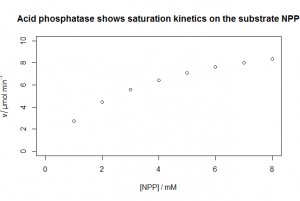The horizontal asymptote is about v=9 or so, and therefore KM (value of [NPP] giving vmax/2) is about 2. The syntax for nls() on this data set is: enzyme.model<-nls( v ~ vmax * S /( KM + S ), data = enzyme.kinetics, start = c( vmax=9, KM=2 ) ) The parameters in the equation you are fitting using the usual ~ tilde syntax can be called whatever you like as long as they meet the usual variable naming conventions for R. The model can be summarised in the usual way: summary( enzyme.model ) Formula: v ~ vmax * S/(KM + S) Parameters: Estimate Std. Error t value Pr(>|t|) vmax 11.85339 0.05618 211.00 7.65e-13 *** KM 3.34476 0.03860 86.66 1.59e-10 *** --- Signif. codes: 0 '***' 0.001 '**' 0.01 '*' 0.05 '.' 0.1 ' ' 1 Residual standard error: 0.02281 on 6 degrees of freedom Number of iterations to convergence: 4 Achieved convergence tolerance: 6.044e-07 The curve-fitting has worked (it has converged after 4 iterations, rather than going into an infinite loop), the estimates of KM and vmax are not far off what we made them by eye, and both are significantly different from zero. If instead you get something like this: Error in nls( y ~ equation.blah.blah.blah, ): singular gradient this means the curve-fitting algorithm has choked and charged off to infinity. You may be able to rescue it by trying nls(…) again, but with different starting estimates. However, there are some cases where the equation simply will not fit (e.g. if the data are nothing like the model you’re trying to fit!) and some pathological cases where the algorithm can’t settle on an estimate. Larger data sets are less likely to have these problems, but sometimes there’s not much you can do aside from trying to fit a simpler equation, or estimating some rough-and-ready parameters by eye. If you do get a model, you can use it to predict the y values for the x values you have, and use that to add a curve of best fit to your data: # Create 100 evenly spaced S values in a one-column data frame headed 'S' predicted.values <- data.frame( S = seq( from = min( enzyme.kinetics$S ),
to         = max( enzyme.kinetics$S ), length.out = 100 ) ) # Use the fitted model to predict the corresponding v values, and assign them # to a second column labelled 'v' in the data frame predicted.values$v <- predict( enzyme.model, newdata=predicted.values )

# Add these 100 x,y data points to the graph, joined up by 99 line-segments
# This will look like a curve at the resolution of the graph
lines( v ~ S, data=predicted.values )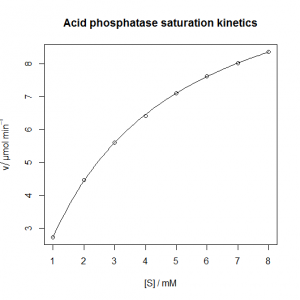If you need to extract a value from the model, you need coef():

vmax.estimate <- coef( enzyme.model )
KM.estimate   <- coef( enzyme.model )

This returns the first and second coefficients out of the model, in the order listed in the summary().

# Exercises

Try fitting non-linear regressions to the data sets below

1. Estimate the doubling time from the bacterial data set in bacterial_growth.csv. N is the optical density of bacterial cells at time t; N0 is the OD at time 0 (you’ll need to estimate N0 too: it’s ‘obviously’ 0.032, but this might have a larger experimental error than the rest of the data set). t is the time (min); and td is the doubling time (min).$N = N_0 e^{ \frac{ \ln{2} }{ t_d } \cdot t }$
1. The response of a bacterium’s growth rate constant μ to an increasing concentration of a toxin, [X], is often well-modelled by a sigmoidal equation of the form below, with a response that depends on the log of the toxin concentration. The file dose_response.csv contains data on the response of the growth constant μ (“mu” in the file, hr‑1) in Enterobacter sp. to the concentration of ammonium ions, [NH4+] (“C” in the file, µM). The graph below shows this data plotted with a nonlinear regression curve. Reproduce it, including the superscripts, Greek letters, italics, etc., in the axis titles; and the smooth curve predicted from the fitted model.$\mu = \frac{ \mu_{max} }{ 1 + e^{ - \frac{ (\ln{[X]} - \ln{IC_{50}} ) }{ s } } }$
• μ is the exponential phase growth constant (hr−1) at any given concentration of X.
• μmax is the maximum value that μ takes, i.e. the value of μ when the concentration of toxin, [X], is zero. The starting estimate should be is whatever the curve seems to be flattening to on the left.
• IC50 is the 50% inhibitory concentration, i.e. the concentration of X needed to reduce μ from μmax to ½μmax. The starting estimate of ln IC50 should be the natural log of the value of [X] that gives you half the maximum growth rate.
• s is the shape parameter: if s is small the curve drops off sharply like a cliff-edge; if s is large, the curve slopes more gently. If s is negative, the curve is Z-shaped; if s is positive, the curve is S-shaped. It starting estimate should be (ln IC25 − ln IC75) / 2, where IC25 is the concentration needed to reduce μ by 25%, and IC75 is the concentration needed to reduce μ by 75%. If you wish to prove this to yourself, pretend that e=3 rather than 2.718… and work out what [X] has to be to give you this value.
• Note the x-variable in the equation and on the plot is the natural logarithm of [X], so you’ll need to use log(C) in your calls to plot() and nls().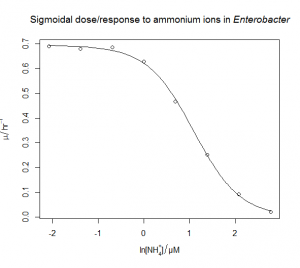1. The bacterium from the previous question is also sensitive to silver(I) ions. The file dose_response_tricky.csv contains μ values (“mu” in the file, hr‑1) in Enterobacter sp. at various concentrations of the silver(I) ion, [Ag+] (“C” in the file, M). Unfortunately, the data does not span all of the interesting part of the response. Try fitting the three-parameter sigmoidal from the previous question. What happens? Can you justify and fit a simpler model?
2. On islands, the number of species tends to increase with the area of the island, but typically as some power-law, rather than proportionally. This is usually modelled with the simple formula shown below, where S is the number of species, A is the area of the island, C is a scaling factor that depends on the choice of area unit, and z is the power-law parameter of interest. Use nonlinear regression to fit this model to the data in carribean_herps.csv (based on Darlington, 1957), which gives the total number of reptile+amphibian (‘herps’) species on seven caribbean islands of varying sizes. You will need to estimate starting parameters for C and z. The traditional way to calculate these parameters is to transform the equation to a linear form using logarithms, and then fit a linear model. You can use this technique to estimate the starting parameters.$S = C A^z$
1. The pH optimum of an enzyme can be modelled by the Michaelis model shown below, where v is the velocity of the enzyme, v0 is the velocity at the poptimum, H is the concentration of hydrogen ions (H = [H+]= 10−pH), and Ka and Kb are two apparent dissociation constants for residues in the active site of the enzyme. ph_optimum.csv gives velocities at various pH values for the enzyme acid phosphatase. v0 is easily eye-balled from the graph. Ka and Kb can also be easily estimated: the line v= v0/2 will intersect the pH dependence curve at two points, one to the left of the optimum, and one to the right: if you drop vertical lines down to the pH axis from each of these two intersections, the left-hand this vertical line cuts the pH axis at the pKa (which is −log10(Ka), and the right-hand oen cuts at the pKb (which is −log10(Kb). Plot the pH dependence curve, and use nonlinear regression to estimate the parameters, and from that the pH optimum, pHopt = −log10(√KaKb).$v = \frac{ v_0 }{ 1 + \frac{H}{K_a} + \frac{K_b}{H} }$

1. The doubling time td is around 25 min.
bacterial.growth<-read.csv( "H:/R/bacterial_growth.csv" )
plot(
N    ~ t,
data = bacterial.growth,
xlab = "N",
ylab = "t / min",
main = "Bacteria grow exponentially"
)

head( bacterial.growth )
   t    N
1  0 0.032
2 10 0.046
…

We estimate the parameters from the plot: N0 is obviously 0.032 from the data above; and the plot (below) shows it takes about 20 min for N to increase from 0.1 to 0.2, so td is about 20 min.

bacterial.model<-nls(
N     ~ N0 * exp( (log(2) / td ) * t),
data  = bacterial.growth,
start = c( N0 = 0.032, td = 20 )
)
summary( bacterial.model )
Formula: N ~ N0 * exp((log(2) / td) * t)
Parameters:
Estimate Std. Error t value Pr(>|t|)
N0 3.541e-02  6.898e-04   51.34 2.30e-11 ***
td 2.541e+01  2.312e-01  109.91 5.25e-14 ***
---
Signif. codes:
0 '***' 0.001 '**' 0.01 '*' 0.05 '.' 0.1 ' ' 1
Residual standard error: 0.002658 on 8 degrees of freedom
Number of iterations to convergence: 5
Achieved convergence tolerance: 1.052e-06

This bit adds the curve to the plot below.

predicted.values <- data.frame(
t = seq(
from       = min( bacterial.growth$t ), to = max( bacterial.growth$t ),
length.out = 100
)
)
predicted.values$N <- predict( bacterial.model, newdata = predicted.values ) lines( N ~ t, data = predicted.values )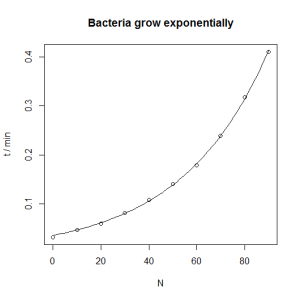1. Dose/response model and graph dose.response<-read.csv( "H:/R/dose_response.csv" ) plot( mu ~ log(C), data = dose.response, xlab = expression("ln" * "[" * NH^"+" * "]" / µM), ylab = expression(mu/hr^-1), main = expression("Sigmoidal dose/response to ammonium ions in " * italic("Enterobacter")) ) The plot indicates that the starting parameters should be • μmax ≈ 0.7 • ln IC50 ≈ ln(3), because IC50 is the value of [X] corresponding to μmax/2 ≈ 0.35, and this is about 3. You can see this by inspecting the raw data (the mid μ value is caused by an [X] somewhere between 2 and 4), or by looking at the plot (where the mid μ value corresponds to a ln([X]) of about 1). We’ll call ln(IC50) logofIC50 in the formula to nls() below so it’s clear this is a parameter we’re estimating, not a function we’re calling. • s ≈ − 0.6, because s ≈ (ln IC25− ln IC75) / 2 ≈ (ln(1.5) − ln(6)) / 2 ≈ (0.4 − 1.8) / 2. IC25 is the concentration needed to reduce μ by 25% (μ = 0.525, corresponding to about 1.5 µM), and IC75 is the concentration needed to reduce μ by 75% (μ = 0.175, corresponding to about 6 µM). dose.model <- nls( mu ~ mumax / ( 1 + exp( -( log(C) - logofIC50 ) / s ) ), data = dose.response, start = c( mumax=0.7, logofIC50=log(3), s=-0.7 ) ) summary( dose.model ) Formula: mu ~ mumax/(1 + exp(-(log(C) - logofIC50)/s)) Parameters: Estimate Std. Error t value Pr(>|t|) mumax 0.695369 0.006123 113.58 1.00e-09 *** logofIC50 1.088926 0.023982 45.41 9.78e-08 *** s -0.509478 0.020415 -24.96 1.93e-06 *** --- Signif. codes: 0 '***' 0.001 '**' 0.01 '*' 0.05 '.' 0.1 ' ' 1 Residual standard error: 0.00949 on 5 degrees of freedom Number of iterations to convergence: 5 Achieved convergence tolerance: 6.394e-06 Achieved convergence tolerance: 5.337e-06 This lists the estimates of μmax, ln(IC50) and s, plus the standard error of these estimates and their p values. Note that they’re all pretty close to what we guessed in the first place, so we can be fairly sure they’re good estimates. To get the actual value of IC50 from the model: exp( coef(dose.model) ) This gives 2.97 µM, which corresponds well with our initial guess. To add the curve, we do the same as before: predicted.values <- data.frame( C = seq( from = min( dose.response$C ),
to         = max( dose.response$C ), length.out = 100 ) ) predicted.values$mu <- predict( dose.model, newdata = predicted.values )
lines( mu ~ log(C), data=predicted.values )

Note that in the real world, you might be hard-pressed to fit a 3-parameter model to a data set of just 8 points, because it is unlikely that they would fall so neatly, and with such a convenient range from zero effect to complete inhibition. In the real world, there’s a very good chance that the nonlinear regression would fail, puking up something like:

Singular gradient


Under these circumstances, you can simplify the model you are trying to fit, e.g. by removing the s parameter (setting it equal to minus 1), which simplifies the equation you are trying to fit to:$\mu = \frac{ \mu_{max} }{ 1 + e^{ (\ln{[X]} - \ln{IC_{50}} ) } }$

and requires this modification to the nls():

dose.model <- nls(
mu    ~ mumax / ( 1 + exp( log(C) - logofIC50 ) ),
data  = dose.response,
start = c( mumax=0.7, logofIC50=log(3) )
)
1. Following on from the previous answer, when we try to fit the silver dose/response, the nonlinear regression fails, even when fed reasonable starting estimates. The solution is to try fitting a simpler model with only two parameters rather than three. We can see what happens if we pass the trace=TRUE argument  to nls(): we can see that the estimates diverge as R tries to fit the curve, rather than converging. One option is to fix mumax at the value obtained from the previous answer. This could be justified if the conditions in the ammonium and the silver(I) growth flasks were otherwise identical. The other option is to fix the value of s at −1 , which has the effect of simplifying the equation as described in the answer above. In both cases, the nonlinear regression now works, and gives similar estimates of IC50: I’d probably choose the second option, but really it would be better overall to collect more data at the low concentration end of the curve if time and materials permitted.
dose.response<-read.csv( "H:/R/dose_response_tricky.csv" )
plot(
mu   ~ log(C),
data = dose.response,
xlab = expression("ln" * "[" * Ag^"+" * "]" / M),
ylab = expression(mu/hr^-1),
main = expression("Dose/response to silver(I) ions in " * italic("Enterobacter"))
)

# Three parameter model

dose.model <- nls(
mu    ~ mumax / ( 1 + exp( -( log(C) - logofIC50 ) / s ) ),
data  = dose.response,
start = c( mumax=0.7, logofIC50=log(1e-5), s=-2 ),
trace=TRUE
)


The output shows divergence (the numbers in the columns are the values of mumax, logofIC50 and s respectively:

0.01784401 :    0.70000 -11.51293  -2.00000
0.01628421 :    0.8985258 -12.5957842  -2.1549230
0.01542858 :    1.061574 -13.223248  -2.218839
0.01507904 :    1.278977 -13.899840  -2.275899
0.01465342 :    1.429477 -14.271384  -2.301364
0.01435196 :    1.610375 -14.662795  -2.325330
0.01419041 :    1.831461 -15.078767  -2.347909
...
Error in nls(mu ~ mumax/(1 + exp(-(log(C) - logofIC50)/s)), data = dose.response,  :
number of iterations exceeded maximum of 50


Option one: fix the value of mumax to the value from the previous answer. This gives us a natural log of IC50 of −11.2386.

# Fix mumax

dose.model.fixed.mumax <- nls(
mu    ~ 0.695 / ( 1 + exp( -( log(C) - logofIC50 ) / s ) ),
data  = dose.response,
start = c( logofIC50=log(1e-5), s=-2 )
)

predicted.values.fixed.mumax <- data.frame(
C = seq(
from       = min( dose.response$C ), to = max( dose.response$C ),
length.out = 100
)
)

predicted.values.fixed.mumax$mu <- predict( dose.model.fixed.mumax, newdata = predicted.values.fixed.mumax ) lines( mu ~ log(C), data=predicted.values.fixed.mumax, col='red' )  Option two: fix the value of s to −1. This gives us a natural log of IC50 of −10.55757. # Fix shape dose.model.fixed.s <- nls( mu ~ mumax / ( 1 + exp( log(C) - logofIC50 ) ), data = dose.response, start = c( mumax=0.7, logofIC50=log(1e-5) ) ) summary( dose.model.fixed.s ) predicted.values.fixed.s <- data.frame( C = seq( from = min( dose.response$C ),
to         = max( dose.response$C ), length.out = 100 ) ) predicted.values.fixed.s$mu <- predict( dose.model.fixed.s,
newdata = predicted.values.fixed.s )

lines( mu ~ log(C), data=predicted.values.fixed.s, col='blue' )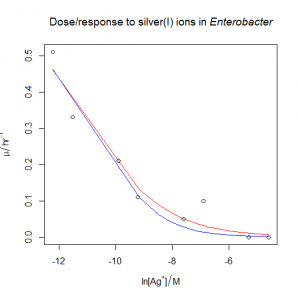1. We use linear regression on the log values to estimate C and z, then we use these values as the starting parameters for the nonlinear regression.
caribbean.herps<-read.csv( "H:/R/caribbean_herps.csv" )

plot(
Species ~ Area,
data = caribbean.herps,
xlab = expression("Area / " * km^2),
ylab = "Number of species of amphibians and reptiles",
main = "Larger islands have more species, but not proportionally so"
)

# Species/area relationship is a power law, so reasonable starting parameters
# can be taken from a log/log plot: if S = CA^z, then log S = log C + z log A

loglog.model<-lm(
log(Species) ~ log(Area),
data = caribbean.herps,
)

# Extract the slope and intercept (named vectors) from the linear model on
# the log/log values, extract the numerical values of these parameters from
# the named vectors, and back-transform the intercept (log C) to get C itself

C.estimate<-exp( as.numeric( coef(loglog.model) ) )
z.estimate<-as.numeric( coef(loglog.model) )

nls.model <- nls(
Species ~ C*Area^z,
data    = caribbean.herps,
start   = c( C=C.estimate, z=z.estimate )
)

summary( nls.model )

predicted.values <- data.frame(
Area = seq(
from       = min( caribbean.herps$Area ), to = max( caribbean.herps$Area ),
length.out = 100
)
)

predicted.values$Species <- predict( nls.model, newdata = predicted.values ) lines( Species ~ Area, data=predicted.values )  The linear model on the log transformed variables gives estimates of C = 2.6 and z=0.30; the nonlinear model on the raw data then gives estimates of C = 1.6 and z=0.35. You’ll note the estimates are rather different.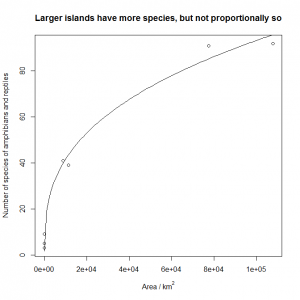1. The optimum is 5.4. ph.optimum<-read.csv( "H:/R/ph_optimum.csv" ) plot( v ~ pH, data = ph.optimum, xlab = "pH", ylab = "v", main = "pH optimum of acid phosphatase" ) ph.model <- nls( v ~ v0 / ( 1 + (10^-pH)/Ka + Kb/(10^-pH) ), data = ph.optimum, start = c( v0=7, Ka=10^-4, Kb=10^-7 ) ) summary( ph.model ) predicted.values <- data.frame( pH = seq( from = min( ph.optimum$pH ),
to         = max( ph.optimum$pH ), length.out = 100 ) ) predicted.values$v <- predict( ph.model, newdata=predicted.values )
lines( v ~ pH, data=predicted.values )

# This calculates the optimum pH:

-log10(sqrt(coef(ph.model)*coef(ph.model)))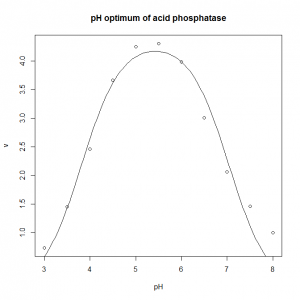## Analysis of variance: ANOVA (2 way)

The technique for a one-way ANOVA can be extended to situations where there is more than one factor, or – indeed – where there are several factors with several levels each, which may have synergistic or antagonistic effects on each other.

In the models we have seen so far (linear regression, one-way ANOVA) all we have really done is tested the difference between a null model (“y is a constant”, “y=“) and a single alternative model (“y varies by group” or “y=a+bx“) using an F test. However, in two-way ANOVA there are several possible models, and we will probably need to proceed through some model simplification from a complex model to a minimally adequate model.

The file wheat_yield.csv contains data on the yield (tn ha−1) of wheat from a large number of replicate plots that were either unfertilised, given nitrate alone, phosphate alone, or both forms of fertiliser. This requires a two-factor two-level ANOVA.

wheat.yield<-read.csv( "H:/R/wheat_yield.csv" )
interaction.plot(
response     = wheat.yield$yield, x.factor = wheat.yield$N,
trace.factor = wheat.yield$P ) As we’re plotting two factors, a box-and-whisker plot would make no sense, so instead we plot an interaction plot. It doesn’t particularly matter here whether we use N(itrate) as the x.factor (i.e. the thing we plot on the x-axis) and P(hosphate) as the trace.factor (i.e. the thing we plot two different trace lines for):You’ll note that the addition of nitrate seems to increase yield: both traces slope upwards from the N(o) to Y(es) level on the x-axis, which represents the nitrate factor. From the lower trace, it appears addition of just nitrate increases yield by about 1 tn ha−1. You’ll also note that the addition of phosphate seems to increase yield: the Y(es) trace for phosphate is higher than the N(o) trace for phosphate. From comparing the upper and lower traces at the left (no nitrate), it appears that addition of just phosphate increases yield by about 2 tn ha−1. Finally, you may notice there is a positive (synergistic) interaction. The traces are not parallel, and the top-right ‘point’ (Y(es) to both nitrate and phosphate) is higher than you would expect from additivity: the top-right is maybe 4, rather than 1+2=3 tn ha−1 higher than the completely unfertilised point. We suspect there is an interaction, this interaction is biologically plausible, and we have 30 samples in each of the four treatments. We fit a two-factor (two-way) ANOVA maximal model, to see whether this interaction is significant. First, we fit the model using N*P to fit the ‘product’ of the nitrate and phosphate factors, i.e. wheat.model<-aov(yield ~ N*P, data=wheat.yield ) anova( wheat.model ) Analysis of Variance Table Response: yield Df Sum Sq Mean Sq F value Pr(>F) N 1 83.645 83.645 43.3631 9.122e-10 *** P 1 256.136 256.136 132.7859 < 2.2e-16 *** N:P 1 11.143 11.143 5.7767 0.01759 * Residuals 136 262.336 1.929 --- Signif. codes: 0 '***' 0.001 '**' 0.01 '*' 0.05 '.' 0.1 ' ' 1 N shows the effect of nitrate, P the effect of phosphate, and N:P is the interaction term. As you can see, this interaction term appears to be significant: the nitrate+phosphate combination seems to give a higher yield than you would expect from just adding up the individual effects of nitrate and phosphate alone. To test this explicitly, we can fit a model that lacks the interaction term, using N+P to fit the ‘sum’ of the factors without the N:P term: wheat.model.no.interaction<-aov(yield ~ N+P, data=wheat.yield ) anova( wheat.model.no.interaction ) Analysis of Variance Table Response: yield Df Sum Sq Mean Sq F value Pr(>F) N 1 83.645 83.645 41.902 1.583e-09 *** P 1 256.136 256.136 128.312 < 2.2e-16 *** Residuals 137 273.479 1.996 --- Signif. codes: 0 '***' 0.001 '**' 0.01 '*' 0.05 '.' 0.1 ' ' 1 We can then use the anova() function with two arguments to compare these two models with an F test: anova( wheat.model, wheat.model.no.interaction ) Analysis of Variance Table Model 1: yield ~ N * P Model 2: yield ~ N + P Res.Df RSS Df Sum of Sq F Pr(>F) 1 136 262.34 2 137 273.48 -1 -11.143 5.7767 0.01759 * --- Signif. codes: 0 '***' 0.001 '**' 0.01 '*' 0.05 '.' 0.1 ' ' 1 An alternative way of doing the same thing is to update the maximal model by deletion. First you fit the maximal model, as before: wheat.model<-aov(yield ~ N*P, data=wheat.yield ) anova( wheat.model ) You note the least significant term (largest p value) is the N:P term, and you selectively remove that from the model to produce a revised model with update(): wheat.model.no.interaction<-update( wheat.model, ~.-N:P) anova( wheat.model, wheat.model.no.interaction ) The ~.-N:P is shorthand for “fit (~) the current wheat.model (.) minus () the interaction term (N:P)”. This technique is particularly convenient when you are iteratively simplifying a model with a larger number of factors and a large number of interactions. Whichever method we use, we note that the deletion of the interaction term significantly reduces the explanatory power of the model, and therefore that our minimally adequate model is the one including the interaction term. If you have larger numbers of factors (say a, b and c) each with a large number of levels (say 4, 2, and 5), it is possible to fit a maximal model (y~a*b*c), and to simplify down from that. However, fitting a maximal model in this case would involve estimating 40 separate parameters, one for each combination of the 4 levels of a, with the 2 levels of b, and the 5 levels of c. It is unlikely that your data set is large enough to make a model of 40 parameters a useful simplification compared to the raw data set itself. It would even saturate it if you have just one datum for each possible {a,b,c} combination. Remember that one important point of a model is to provide a simplification of a large data set. If you want to detect an interaction between factors a and b reliably, you need enough data to do so. In an experimental situation, this might impact on whether you actually want to make c a variable at all, or rather to control it instead, if this is possible. # Exercises Analyse the following data set with ANOVA 1. The file glucose_conc.csv contains fasting blood serum glucose levels (mg dL−1) for humans homozygous for either a mutant allele or the wild-type allele at two loci (Rtfm and Stfw) thought to be involved in blood glucose control. Do the data support a correlation between possession of the mutant alleles and elevated glucose levels? # Answers 1. Glucose concentrations glucose.conc<-read.csv( "H:/R/glucose_conc.csv" ) interaction.plot( response = glucose.conc$conc,
x.factor     = glucose.conc$Stfw, trace.factor = glucose.conc$Rtfm
)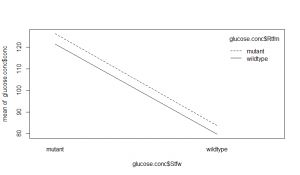The lines are parallel, so there seems little evidence of interaction between the loci. The difference between the mutant and wildtypes for the Rtfm locus doesn’t look large, and may not be significant. However, the Stfw wildtypes seem to have better control of blood glucose. A two-way ANOVA can investigate this:

glucose.model<-aov( conc ~ Stfw*Rtfm, data = glucose.conc )
anova( glucose.model )
Analysis of Variance Table
Response: conc
Df Sum Sq Mean Sq  F value  Pr(>F)
Stfw        1 133233  133233 311.4965 < 2e-16 ***
Rtfm        1   1464    1464   3.4238 0.06526 .
Stfw:Rtfm   1     17      17   0.0407 0.84020
Residuals 296 126605     428
---
Signif. codes:
0 '***' 0.001 '**' 0.01 '*' 0.05 '.' 0.1 ' ' 1

As we suspected, the Stfw:Rtfm interaction is not significant. We will remove it by deletion:

glucose.model.no.interaction<-update( glucose.model, ~.-Stfw:Rtfm )
anova( glucose.model, glucose.model.no.interaction )
Analysis of Variance Table

Model 1: conc ~ Stfw * Rtfm
Model 2: conc ~ Stfw + Rtfm
Res.Df    RSS Df Sum of Sq      F Pr(>F)
1    296 126605
2    297 126622 -1   -17.421 0.0407 0.8402

The p value is much larger than 0.05, so the model including the interaction term is not significantly better than the one excluding it.

The reduced model is now:

anova(glucose.model.no.interaction )
Analysis of Variance Table
Response: conc
Df Sum Sq Mean Sq  F value  Pr(>F)
Stfw        1 133233  133233 312.5059 < 2e-16 ***
Rtfm        1   1464    1464   3.4349 0.06482 .
Residuals 297 126622     426
---
Signif. codes:
0 '***' 0.001 '**' 0.01 '*' 0.05 '.' 0.1 ' ' 1

When we remove the interaction term, its variance is redistributed to the remaining factors, which will change their values compared to the maximal model we fitted at the start. It appears that the wildtype and mutants of Rtfm do not significantly differ in their glucose levels, so we will now remove that term too:

glucose.model.stfw.only<-update( glucose.model.no.interaction, ~.-Rtfm )
anova( glucose.model.no.interaction, glucose.model.stfw.only )
Analysis of Variance Table
Model 1: conc ~ Stfw + Rtfm
Model 2: conc ~ Stfw
Res.Df    RSS Df Sum of Sq      F  Pr(>F)
1    297 126622
2    298 128087 -1   -1464.4 3.4349 0.06482 .
---
Signif. codes:
0 '***' 0.001 '**' 0.01 '*' 0.05 '.' 0.1 ' ' 1

Again, we detect no significant different between the models, so we accept the simpler one (Occam’s razor).

anova( glucose.model.stfw.only )
Analysis of Variance Table

Response: conc
Df Sum Sq Mean Sq F value    Pr(>F)
Stfw        1 133233  133233  309.97 < 2.2e-16 ***
Residuals 298 128087     430
---
Signif. codes:
0 '***' 0.001 '**' 0.01 '*' 0.05 '.' 0.1 ' ' 1

The Stfw locus does have a very significant effect on blood glucose levels. We could try deletion testing Stfw too, but here it in not really necessary as the ANOVA table above is comparing the Stfw only model to the null model in any case. To determine what the effect of Stfw is, we can use a Tukey test:

TukeyHSD( glucose.model.stfw.only )
  Tukey multiple comparisons of means
95% family-wise confidence level
Fit: aov(formula = conc ~ Stfw, data = glucose.conc)
$Stfw diff lwr upr p adj wildtype-mutant -42.14783 -46.859 -37.43666 0 … or, as this model has in fact simplified down to the two-levels of one factor, this is equivalent to just doing a t test at this point: t.test( conc ~ Stfw, data = glucose.conc)  Welch Two Sample t-test data: conc by Stfw t = 17.6061, df = 289.288, p-value < 2.2e-16 alternative hypothesis: true difference in means is not equal to 0 95 percent confidence interval: 37.43609 46.85958 sample estimates: mean in group mutant mean in group wildtype 123.87824 81.73041 Given this analysis, the best way to represent our data is probably a simple boxplot ignoring Rtfm, with an explanatory legend: boxplot( conc ~ Stfw, data = glucose.conc, xlab = expression( italic(Sftw) ), ylab = expression( "[Glucose] / mg "*dL^-1 ), main = "Mutants at the Stfw locus are less able\nto control their blood glucose levels" )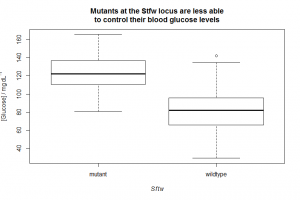Homozygous mutants at the Stfw locus were found to be less well able to control their blood glucose levels (F=310, p≪0.001). Homozygous mutation of the Rtfm locus was found to have no significant effect on blood glucose levels (F on deletion from ANOVA model = 3.4, p=0.06). Homozygous mutation at the Stfw locus was associated with a blood glucose level 40 mg dL−1 (95% CI: 37.4…46.8) higher than the wildtype (t=17.6, p≪0.001). Next up… Nonlinear regression. ## Analysis of variance: ANOVA (1 way) Analysis of variance is the technique to use when you might otherwise be considering a large number of pairwise F and t tests, i.e. where you want to know whether a factor with more than 2 levels is a useful predictor of a dependent variable. For example, cuckoo_eggs.csv contains data on the length of cuckoo eggs laid into different host species, the (meadow) pipit, the (reed) warbler, and the wren. A box-and-whisker plot is a useful way to view data of this sort: cuckoo.eggs<-read.csv( "H:/R/cuckoo_eggs.csv" ) boxplot( egg.length ~ species, data = cuckoo.eggs, xlab = "Host species", ylab = "Egg length / mm" )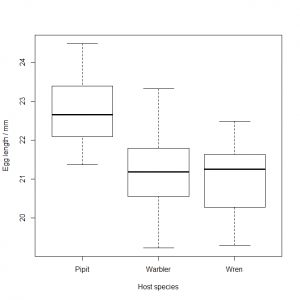You might be tempted to try t testing each pairwise comparison (pipit vs. wren, warbler vs. pipit, and warbler vs. wren), but a one-factor analysis of variance (ANOVA) is what you actually want here. ANOVA works by fitting individual means to the three levels (warbler, pipit, wren) of the factor (host species) and seeing whether this results in a significantly smaller residual variance than fitting a simple overall mean to the entire data set. Conceptually, this is very similar to what we did with linear regression: ANOVA compares the residuals on the model represented by the “y is a constant” graph below: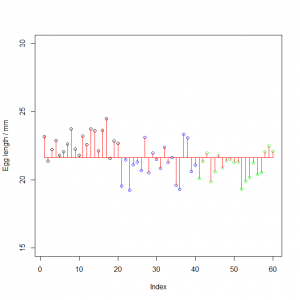…with a model where three individual means have been fitted, the “y varies by group” model: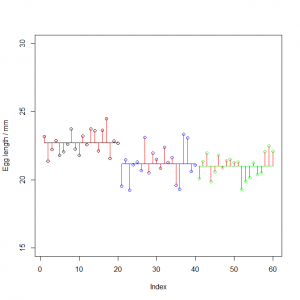It’s not immediately obvious that fitting three separate means has bought us much: the model is more complicated, but the length of the red lines doesn’t seem to have changed hugely. However, R can tell us precisely whether or not this is the case. The syntax for categorical model fitting is aov(), for analysis of variance: aov( egg.length ~ species, data=cuckoo.eggs ) Call: aov(formula = egg.length ~ species, data = cuckoo.eggs) Terms: species Residuals Sum of Squares 35.57612 55.85047 Deg. of Freedom 2 57 Residual standard error: 0.989865 Estimated effects may be unbalanced As with linear regression, you may well wish to save the model for later use. It is traditional to display the results of an ANOVA in tabular format, which can be produced using anova() cuckoo.model<-aov( egg.length ~ species, data=cuckoo.eggs ) anova( cuckoo.model ) Analysis of Variance Table Response: egg.length Df Sum Sq Mean Sq F value Pr(>F) species 2 35.576 17.7881 18.154 7.938e-07 *** Residuals 57 55.850 0.9798 --- Signif. codes: 0 ‘***’ 0.001 ‘**’ 0.01 ‘*’ 0.05 ‘.’ 0.1 ‘ ’ 1 The “y is a constant” model has only one variance associated with it: the total sum of squares of the deviances from the overall mean (SST, or SSY, whichever you prefer) divided by the degrees of freedom in the data set (n−1). The “y varies by group” model decomposes this overall variance into two components. • The first component (SSA) is associated with categorising the data into k groups (k=3 here). It is the sum of squares of the difference between the group-specific mean of each data point from the overall mean, divided by the number of degrees of freedom those k means have, (k−1). This represents the between group variation in the data. • The second component (SSE) is the residual variance, left unexplained by assigning k different means to each of the k groups. It is the sum of squares of the data points from their group-specific mean, divided by the degrees of freedom left after estimating those k individual means, (nk). This represents the within group variation in the data. In the ANOVA table, the Sum Sq is the sum of the squares of the deviances of the data points from a mean. The Df is the degrees of freedom, and the Mean Sq is the sum of squares divided by the degrees of freedom, which is the corresponding variance. The F value is the mean-square for species divided by the mean-square of the Residuals. The p value indicates that categorising the data into three groups does make a significant difference to explaining the variance in the data, i.e. estimating three separate mean for each host species, rather than one grand mean, does make a significant difference to how well we can explain the data. The length of the eggs the cuckoo lays does vary by species. Compare this with linear regression, where you’re trying to find out whether y=a+bx is a better model of the data than y=. This is very similar to ANOVA, where we are trying to find out whether “y varies by group, i.e. the levels of a factor” is a better model than “y is a constant, i.e. the overall mean”. You might well now ask “but which means are different?” This can be investigated using TukeyHSD() (honest significant differences) which performs a (better!) version of the pairwise t test you were probably considering at the top of this post. TukeyHSD( cuckoo.model )  Tukey multiple comparisons of means 95% family-wise confidence level Fit: aov(formula = egg.length ~ species, data = cuckoo.eggs)$species
Warbler-Pipit -1.5395 -2.2927638 -0.7862362 0.000023
Wren-Pipit    -1.7135 -2.4667638 -0.9602362 0.000003
Wren-Warbler  -0.1740 -0.9272638  0.5792638 0.843888

This confirms that – as the box-and-whisker plots suggested – the eggs laid in wren and warbler nests are not significantly different in size, but those laid in pipit nests are significantly larger than those in the warbler or wren nests.

ANOVA has the same sorts of assumption as the F and t tests and as linear regression: normality of residuals, homoscedacity, representativeness of the sample, no error in the treatment variable, and independence of data points. You should therefore use the same checks after model fitting as you used for linear regression:

plot( residuals( cuckoo.model ) ~ fitted( cuckoo.model ) )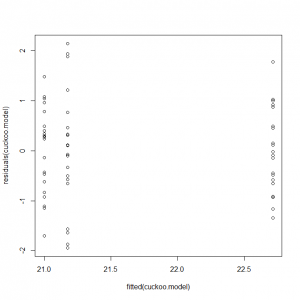We do not expect a starry sky in the residual plot, as the fitted data are in three discrete levels. However, if the residuals are homoscedastic, we expect them to be of similar spread in all three treatments, i.e. the residuals shouldn’t be more scattered around the zero line in the pipits than in the wrens, for example. This plot seems consistent with that.

qqnorm( residuals( cuckoo.model ) )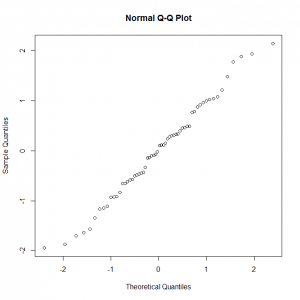On the normal Q-Q plot, we do expect a straight line, which – again – we appear to have (although it’s a bit jagged, and a tiny bit S-shaped). We can accept that the residuals are more-or-less normal, and therefore that the analysis of variance was valid.

# Exercises

Analyse the following data set with ANOVA

1. The file venus_flytrap.csv contains data on the wet biomass (g) of Venus flytraps prevented from catching flies (control), allowed to catch flies, or prevented from catching flies but instead given fertiliser applied to the soil. Does the feeding treatment significantly affect the biomass? If so, which of the three means differ significantly, and in what directions? Have a look at the residuals in the same way as you did for the regression. How do they look?

1. Venus flytrap biomass data
venus.flytrap<-read.csv("H:/R/venus_flytrap.csv")
plot(
biomass ~ feed,
data = venus.flytrap,
xlab = "Feeding treatment",
ylab = "Wet biomass / g",
main = expression("Venus flytrap feeding regime affects wet biomass")
)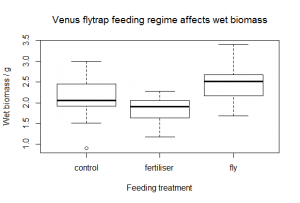flytrap.model<-aov( biomass ~ feed, data = venus.flytrap )
anova( flytrap.model )
Analysis of Variance Table
Response: biomass
Df  Sum Sq Mean Sq F value    Pr(>F)
feed      2  5.8597 2.92983  22.371 1.449e-08 ***
Residuals 87 11.3939 0.13096
---
Signif. codes:
0 '***' 0.001 '**' 0.01 '*' 0.05 '.' 0.1 ' ' 1
TukeyHSD( flytrap.model )
  Tukey multiple comparisons of means
95% family-wise confidence level
Fit: aov(formula = biomass ~ feed, data = venus.flytrap)
$feed diff lwr upr p adj fertiliser-control -0.3086667 -0.53147173 -0.0858616 0.0039294 fly-control 0.3163333 0.09352827 0.5391384 0.0030387 fly-fertiliser 0.6250000 0.40219493 0.8478051 0.0000000 The feeding treatment makes a significant different to the wet biomass (F=22.3, p=1.45 × 10−8) and a Tukey HSD test shows that all three means are different, with the fly treatment having a beneficial effect (on average, the fly-treated plants are 0.32 g heavier than the control plants), but the fertiliser has an actively negative effect on growth, with these plants on average being 0.31 g lighter than the control plants. To plot the residuals, we use the same code as for the linear regression: plot( residuals(flytrap.model) ~ fitted(flytrap.model) )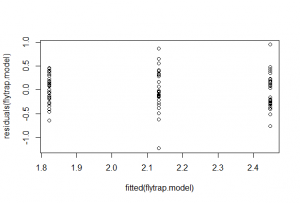There is perhaps a bit more scatter in the residuals of the control (the ones in the middle), but nothing much to worry about. qqnorm( residuals( flytrap.model ) )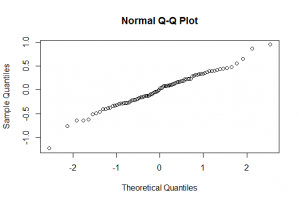On the normal Q-Q plot, we do expect a straight line, which – again – we appear to have. We can accept that the residuals are essentially homoscedastic and normal, and therefore that the analysis of variance was valid. Next up… Two-way ANOVA. ## Comparison of expected and observed count data: the χ² test A χ2 test is used to measure the discrepancy between the observed and expected values of count data. • The dependent data must – by definition – be count data. • If there are independent variables, they must be categorical. The test statistic derived from the two data sets is called χ2, and it is defined as the square of the discrepancy between the observed and expected value of a count variable divided by the expected value.$\chi^2 = \sum{ \frac{ (O - E)^2 }{ E } }$ The reference distribution for the χ2 test is Pearson’s χ2. This reference distribution has a single parameter: the number of degrees of freedom remaining in the data set. A χ2 test compares the χ2 statistic from your empirical data with the Pearson’s χ2 value you’d expect under the null hypothesis given the degrees of freedom in the data set. The p value of the test is the probability of obtaining a test χ2 statistic at least as extreme as the one that was actually observed, assuming that the null hypothesis (“there is no discrepancy between the observed and expected values”) is true. i.e. The p value is the probability of observing your data (or something more extreme), if the data do not truly differ from your expectation. The comparison is only valid if the data are: • Representative of the larger population, i.e.the counts are sampled in an unbiased way. • Of sufficiently large sample size. In general, observed counts (and expected counts) less than 5 may make the test unreliable, and cause you to accept the null hypothesis when it is false (i.e. ‘false negative’). R will automatically apply Yates’ correction to values less than 5, but will warn you if it thinks you’re sailing too close to the wind. • Independent. Do not use a χ2 test unless these assumptions are met. The Fisher’s exact test fisher.test() may be more suitable if the data set is small. In R, a χ2-test is performed using chisq.test(). This acts on a contingency table, so the first thing you need to do is construct one from your raw data. The file tit_distribution.csv contains counts of the total number of birds (the great tit, Parus major, and the blue tit, Cyanistes caeruleus) at different layers of a canopy over a period of one day. tit.distribution<-read.csv( "H:/R/tit_distribution.csv" ) print( tit.distribution ) This will spit out all 706 observations: remember that the raw data you import into R should have a row for each ‘individual’, here each individual is a “This bird in that layer” observation. You can see just the start of the data using head(): head( tit.distribution )  Bird Layer 1 Bluetit Ground 2 Bluetit Ground 3 Bluetit Ground 4 Bluetit Ground 5 Bluetit Ground 6 Bluetit Ground and look at a summary of the data frame object with str(): str( tit.distribution ) 'data.frame': 706 obs. of 2 variables:$ Bird :
Factor w/ 2 levels "Bluetit","Greattit": 1 1 1 1 1 1 1 1 1
1 ...
$Layer: Factor w/ 3 levels "Ground","Shrub",..: 1 1 1 1 1 1 1 1 1 1 ... To create a contingency table, use table(): tit.table<-table( tit.distribution$Bird, tit.distribution$Layer ) tit.table  Ground Shrub Tree Bluetit 52 72 178 Greattit 93 247 64 If you already had a table of the count data, and didn’t fancy making the raw data CSV file from it, just to have to turn it back into a contingency table anyway, you could construct the table manually using matrix(): tit.table<-matrix( c( 52, 72, 178, 93, 247, 64 ), nrow=2, byrow=TRUE ) # nrows means cut the vector into two rows # byrow=TRUE means fill the data in horizontally (row-wise) # rather than vertically (column-wise) tit.table  [,1] [,2] [,3] [1,] 52 72 178 [2,] 93 247 64 The matrix can be prettified with labels (if you wish) using dimnames(), which expects a list() of two vectors, the first of which are the row names, the second of which are the column names: dimnames( tit.table )<-list( c("Bluetit","Greattit" ), c("Ground","Shrub","Tree" ) ) tit.table  Ground Shrub Tree Bluetit 52 72 178 Greattit 93 247 64 To see whether the observed values (above) differ from the expected values, you need to know what those expected values are. For a simple homogeneity χ2test, the expected values are simply calculated from the corresponding column (C), row (R) and grand (N) totals:$E = \frac{R \times C}{ N }$  Ground Shrub Tree Row totals Blue tit 52 72 178 302 E 302×145/706 = 62.0 302×319/706 = 136.5 302×242/706 = 103.5 χ2 (52−62)2/62 = 1.6 (72−136.5)2/136.5 = 30.5 (178−103.5)2/103.5 = 53.6 Great tit 93 247 64 404 E 404×145/706 = 83.0 404×319/706 = 182.5 404×242/706 = 138.5 χ2 (93−83)2/83 = 1.2 (247−182.5)2/182.5 = 22.7 (64−138.5)2/138.5 = 40.1 Column totals 145 319 242 706 The individual χ2 values show the discrepancies for each of the six individual cells of the table. Their sum is the overall χ2 for the data, which is 149.7. R does all this leg-work for you, with the same result: chisq.test( tit.table )  Pearson's Chi-squared test data: tit.table X-squared = 149.6866, df = 2, p-value < 2.2e-16 The individual tits’ distributions are significantly different from homogeneous, i.e. there are a lot more blue tits in the trees and great tits in the shrub layer than you would expect just from the overall distribution of birds. Sometimes, the expected values are known, or can be calculated from a model. For example, if you have 164 observations of progeny from a dihybrid selfing genetic cross, where you expect a 9:3:3:1 ratio, you’d perform a χ2 manually like this:  A- B- A- bb aa B- aa bb O 94 33 28 9 E 164×9/16 = 92.25 164×3/16 = 30.75 164×3/16 = 30.75 164×1/16 = 10.25 χ2 (94−92.25)2/92.25 = 0.033 (33−30.75)2/30.75 = 0.165 (28−30.75)2/30.75 = 0.246 (9−10.25)2/10.25 = 0.152 For a total χ2of 0.596. To do the equivalent in R, you should supply chisq.test() with a second, named parameter called p, which is a vector of expected probabilities: dihybrid.table<-matrix( c( 94, 33, 28, 9 ), nrow=1, byrow=TRUE ) dimnames( dihybrid.table )<-list( c( "Counts" ), c( "A-B-","A-bb","aaB-","aabb" ) ) dihybrid.table  A-B- A-bb aaB- aabb Counts 94 33 28 9 null.probs<-c( 9/16, 3/16, 3/16, 1/16 ) chisq.test( dihybrid.table, p=null.probs )  Chi-squared test for given probabilities data: dihybrid.table X-squared = 0.5962, df = 3, p-value = 0.8973 The data are not significantly different from a 9:3:3:1 ratio, so the A and B loci appear to be unlinked and non-interacting, i.e. they are inherited in a Mendelian fashion. The most natural way to plot count data is using a barplot() bar-chart: barplot( dihybrid.table, xlab="Genotype", ylab="N", main="Dihybrid cross" )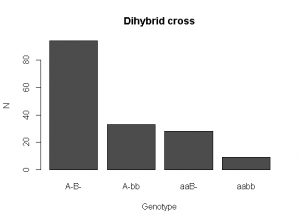# Exercises Use the χ2 test to investigate the following data sets. 1. Clover plants can produce cyanide in their leaves if they possess a particular gene. This is thought to deter herbivores. Clover seedlings of the CN+ (cyanide producing) and CN (cyanide free) phenotypes were planted out and the amount of rabbit nibbling to leaves was measured after 48 hr. Leaves with >10% nibbling were scored as ‘nibbled’, those with less were scored as ‘un-nibbled’. Do the data support the idea that cyanide reduces herbivore damage?  Nibbled Un-nibbled CN+ 26 74 CN− 34 93 1. In a dihybrid selfing cross between maize plants heterozygous for the A/a (A is responsible for anthocyanin production) and Pr/pr (Pr is responsible for modification of anthocyanins from red to purple) loci, we expect an F2 ratio of 9 A− Pr−: 3 A− pr pr: 3 a a Pr− : 1 a a pr pr. The interaction between the loci results in the a a Pr− and a a pr pr individuals being indistinguishable in the colour of their kernels. The file maize_kernels.csv contains a tally of kernel colours. Do the data support the gene-interaction model? # Answers 1. As the data is already in a table, it is easier to construct it directly as a matrix. The data do not support the hypothesis that the two phenotype differ in their damage from rabbit nibbling clover.table<-matrix( c( 26, 74, 34, 93 ), nrow=2, byrow=TRUE ) dimnames( clover.table )<-list( c( "CN.plus", "CN.minus" ), c( "Nibbled", "Un.nibbled" ) ) clover.table  Nibbled Un.nibbled CN.plus 26 74 CN.minus 34 93 chisq.test( clover.table )  Pearson's Chi-squared test with Yates' continuity correction data: clover.table X-squared = 0, df = 1, p-value = 1 1. The data are in a file, so we construct a simple contingency table from that and then test against the expected frequencies of 9 purple : 3 red : 4 colourless. Make sure you get them in the right order! The data support the model, as the χ2 value has a p value greater than 0.05, i.e. we can accept that the data are consistent with a 9:3:4 ratio. maize.kernels<-read.csv( "H:/R/maize_kernels.csv" ) head( maize.kernels )  Kernel 1 Red 2 Colourless 3 Colourless 4 Colourless 5 Purple 6 Colourless maize.table<-table( maize.kernels$Kernel )
maize.table
Colourless    Purple        Red
229       485        160
chisq.test( maize.table, p=c( 4/16, 9/16, 3/16 ) )
Chi-squared test for given probabilities
data: maize.table
X-squared = 0.6855, df = 2, p-value = 0.7098

Next up… One-way ANOVA.

## Correlation of data: linear regression

Linear regression is used to see whether one continuous variable is correlated with another continuous variable in a linear way, i.e. can the dependent variable y be modelled with a straight-line response to changes in the independent covariate x:$y = a + bx + \epsilon$

Here b is the estimated slope of the best-fit line (a.k.a. gradient, often written m), a is its y-intercept (often written c), and ϵ is the residual error. If the x and y data are perfectly correlated, then ϵ=0 for each and every x,y pair in the in the data set; however, this is extremely unlikely to occur in real-world data.

When you fit a linear model like this to a data set, each coefficient you fit (here, the intercept and the slope) will be associated with a t value and p value, which are essentially the result of a one-sample t test comparing the fitted value to 0.

Linear regression is only valid if:

• The x and y variables have a linear relationship. If y is a function of the square of x, or has a hyperbolic relationship with x, then naïve linear regression must not be used, as it will try to fit a straight line to what is clearly not a straight-line relationship. It is often possible to transform curved relationships to straight-line relationships using transformation (logs, reciprocals, etc.) Always plot() and eyeball your data before modelling! A salutary warning of what happens when you don’t plot your data first is Anscombe’s Quartet.
• The data sets are representative of the larger population. As usual, if you collect data that is biased, fraudulent or of very small size, then any kind of statistical analysis is likely to be broken.
• The residuals are normally distributed and homoscedastic. When the linear model is fitted, there will be some residual ‘noise’, i.e. the ϵ error term above. These residuals must be normally distributed, and should not be a function of the value of the x variable, i.e. the variance of y data at small values of x should be the same as the variance of y data at large values of x.
• Each pair of data is independent. Each x,y pair should be independent of every other x,y pair.
• The x variable is measured without error. Only the y variable can have an error associated with it.

Linear regression is very commonly used in circumstances where it is not technically appropriate, e.g. time-series data (where later x,y pairs are most certainly not independent of earlier pairs), or where the x-variable does have some error associated with it (e.g. from pipetting errors), or where a transformation has been used that will make the residuals non-normal. You should at least be aware you are breaking the assumptions of the linear regression procedure if you use it for data of this sort.

The file cricket_chirps.csv contains data on the frequency of cricket chirps (Hz) at different temperatures (°C). A quick plot of the data seems to show a positive, linear relationship:

cricket.chirps<-read.csv( "H:/R/cricket_chirps.csv" )
plot(
Frequency ~ Temperature,
data = cricket.chirps,
xlab = "Temperature / °C",
ylab = "Frequency / Hz",
main ="Crickets chirp more frequently at higher temperatures",
pch  = 15
# The pch option can be used to control the pointer character
)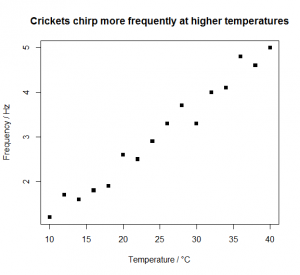To model the data, you need to use lm( y ~ x, data=data.frame ). The lm() stands for “linear model”.

lm( Frequency ~ Temperature, data=cricket.chirps )
Call:
lm(formula = Frequency ~ Temperature, data = cricket.chirps)
Coefficients:
(Intercept)       Temperature
-0.1140       0.1271

You’ll often want to save the model for later use, so you can assign it to a variable. summary() can then be used to see what R thinks the slope and intercept are:

chirps.model<-lm( Frequency ~ Temperature, data=cricket.chirps )
summary( chirps.model )
Call:
lm(formula = Frequency ~ Temperature, data=cricket.chirps)

Residuals:
Min       1Q    Median       3Q       Max
-0.39779 -0.11544  -0.00191  0.12603   0.33985

Coefficients:

Estimate Std. Error t value Pr(>|t|)
(Intercept) -0.113971   0.152264  -0.749    0.467
Temperature  0.127059   0.005714  22.235 2.55e-12 ***
---
Signif. codes:
0 '***' 0.001 '**' 0.01 '*' 0.05 '.' 0.1 ' ' 1
Residual standard error: 0.2107 on 14 degrees of freedom
Multiple R-squared:
F-statistic: 494.4 on 1 and 14 DF,  p-value: 2.546e-12
• The (Intercept) is a from the formula at the top of this section. It is the y-intercept of the line of best fit through the x,y data pairs. It is value of y (Frequency) when x (Temperature) is zero, i.e. how frequently the crickets chirp at the freezing point of water. The estimated value is -0.1140 Hz, which is impossible(!), but satisfyingly does not appear to be significantly different from zero (p=0.467).
• The Temperature is b from the formula at the top of this section. It is the slope of line of best fit through the x,y data pairs. The estimated value is 0.1271 Hz °C−1, i.e. for every 10°C increase in temperature, the chirping rate increases by about 1.3 Hz.
• The Multiple R-squared value is the square of the correlation coefficient R for Frequency on Temperature. Values of R2 close to 1 indicate y is well correlated with the x covariate with relatively little scatter; values close to 0 indicate the scatter is large and x is a poor predictor of y.

To understand the meaning of the F-statistic part of the report, it is important to understand what actually happens when you perform a linear regression. What you’re really trying to find out in linear regression is whether a straight-line with non-zero slope “y=a+bx” is a better model of the dependent variable than a straight line of slope zero, with a y-intercept equal to a constant, usually the mean of the y values: “y=“. These two possible models are shown below. The code needed to display them with the deviance of each datum from the regression line picked out as red (col="red") vertical line segments is also shown.

Linear regression model “y=a+bx” with non-zero slope…

chirps.model<-lm( Frequency ~ Temperature, data=cricket.chirps )
abline( chirps.model )
chirps.predicted<-data.frame( Temperature=cricket.chirps$Temperature ) chirps.predicted$Frequency<-predict( chirps.model, newdata=chirps.predicted )
segments(
cricket.chirps$Temperature, cricket.chirps$Frequency,
chirps.predicted$Temperature, chirps.predicted$Frequency,
col="red"
)

We use the predict() function to predict the Frequency values from the model and a data frame containing Temperature values. We put the predicted Frequency values into a new column in the data frame by assigning them using the $ dollar syntax. To add a regression line to the current plot using the fitted model, we use abline(). Like many of the other functions we’ve seen, this can either take an explicit intercept and slope: # abline( a=intercept, b=slope ) abline( a=-0.1140, b=0.1271 ) Or it can take a tilde ~ modelled-by formula: abline( cricket.chirps.model ) We use the segments() function to add red line segments to represent the deviation of each datum from the regression line. segments() takes four vectors as arguments, the x and y coordinates to start each segment from (here, the measured Temperature, Frequency data points), plus the x and y coordinates to finish each line (the equivalent columns from the data frame containing the predicted data: these are the corresponding points on the regression line).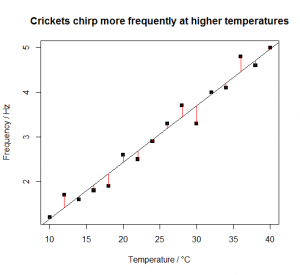y is a constant “y= ” model with zero slope… mean.frequency<-mean( cricket.chirps$Frequency )
abline( a=mean.frequency, b=0 )
segments(
cricket.chirps$Temperature, cricket.chirps$Frequency,
cricket.chirps$Temperature, rep( mean.frequency, length(cricket.chirps$Frequency) ),
col="red"
)

The constant is the mean of the Frequency measurements. The predicted Frequency values are therefore just 16 copies of this mean. We use length() to avoid having to hard-code the ’16’.It is ‘obvious’ that the y=a+bx model is better than the y= model. The y= model estimates just one parameter from the data (the mean of y), but leaves a huge amount of residual variance unexplained. The y=a+bx model estimates one more parameter, but with an enormous decrease in the residual variance, and a correspondingly enormous increase in the model’s explanatory power.

How much better? The degree to which the y=a+bx model is better than the y= model is easily quantified using an F test, and in fact R has already done this for you in the output from summary( chirps.model ):

F-statistic: 494.4 on 1 and 14 DF,  p-value: 2.546e-12

Accounting for the covariate Temperature makes a significant difference to our ability to explain the variance in the Frequency values. The F statistic is the result of an F test comparing the residual variance in the y=a+bx model (i.e. the alternative hypothesis: “Temperature makes a difference to frequency of chirps”) with the residual variance the y= model (i.e. the null hypothesis “Temperature makes no difference to frequency of chirps”).

An F test tells you whether two variances are significantly different: these can be the variances of two different data sets, or – as here – these can be the residual variances of two different models. The F value is very large (494) and the difference in explanatory power of the two models is therefore significantly different: by estimating just one extra parameter, the slope, which requires us to remove just one extra degree of freedom, we can explain almost all of the variance in the data.

Once we have fitted a linear model, we should check that the fit is good and that the assumption about the normality of the residual variance in the y variable is satisfied.

plot( residuals(chirps.model) ~ fitted(chirps.model) )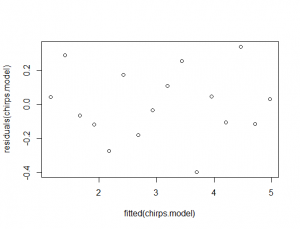This plots the fitted Frequency values (i.e. Frequency.fitted = 0.1271×Temperature-0.1140) as the x variable against the residual values (ε=Frequency-Frequency.fitted) as the y variable. If the residuals are behaving themselves (i.e. they are normal), this should look like a starry sky, with equal numbers of points above and below 0. If the residuals increase or decrease (i.e. it looks like you could stick a sloping line or a curve through them) with the fitted values, or are asymmetrically distributed, then your data break the assumptions of linear regression, and you should be careful in their interpretation.

You should also look at the normal quantile-quantile (QQ) plot of the residuals:

qqnorm( residuals( chirps.model ) )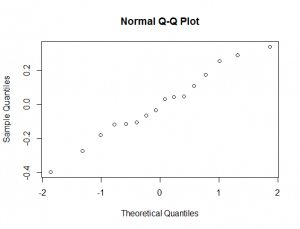The points on this graph should lie on a straight line. If they’re curved, again, your data break the assumptions of linear regression, and you should be careful in their interpretation. You can scan through these and other diagnostic plots using:

plot( chirps.model )

# Exercises

Fit linear models to the following data sets. Criticise the modelling: are the assumptions of the linear regression met?

1. Using the sycamore_seeds.csv file you have already made, model the data and add a suitable regression line to the graph. You saved the code for the graph, and the CSV file from before, didn’t you?
2. The file nadh_absorbance.csv contains data on the absorbance (A) at 340 nm of solutions containing increasing micromolar concentrations (C) of NADH. What is the Beer-Lambert molar extinction coefficient (ϵ) for NADH at 340 nm? How confident are you in your estimate? Is there anything about the data set that violates the linear regression assumptions? [Note that the epsilon here is the standard symbol for molar extinction coefficient and has nothing to do with the residuals]$A=\epsilon C l$
1. There is a relationship between the size of an island (or other defined area) and the number of species it contains. This relationship is modelled by the equation below, where S is the number of species, A is the area, and C and z are data-specific constants. Using logs, convert this equation into a linear form. Use R to transform the data below, and to estimate C and z. Criticise your model, and comment on the reliability of the results.$S=C A^z$
 Island Area of island / km2 Number of (non-bat) mammal species Jersey 116.3 9 Guernsey 63.5 5 Alderney 7.9 3 Sark 5.2 2 Herm 1.3 2

1. Sycamore seeds regression
sycamore.seeds<-read.csv( "H:/R/sycamore_seeds.csv" )
plot(
descent.speed ~ wing.length,
data = sycamore.seeds,
xlab = "Wing length / mm",
ylab = expression("Descent speed " / m*s^-1),
main = "Sycamore seeds with longer wings fall more slowly"
)
sycamore.seeds.model<-lm( descent.speed ~ wing.length, data=sycamore.seeds )
abline( sycamore.seeds.model )
summary( sycamore.seeds.model )
Call:
lm(formula = descent.speed ~ wing.length, data = sycamore.seeds)
Residuals:
Min        1Q   Median        3Q       Max
-0.073402 -0.034124 -0.005326  0.005395 0.105636
Coefficients:
Estimate Std. Error t value Pr(>|t|)
(Intercept)  2.388333   0.150479  15.872 9.56e-07 ***
wing.length -0.040120   0.004607  -8.709 5.28e-05 ***
---
Signif. codes:
0 '***' 0.001 '**' 0.01 '*' 0.05 '.' 0.1 ' ' 1
Residual standard error: 0.06416 on 7 degrees of freedom
Multiple R-squared:
R-squared:  0.9034
F-statistic: 75.85 on 1 and 7 DF,  p-value: 5.28e-05

Both the intercept and the slope are significantly different from zero. The slope is negative, around −0.04 m s−1 mm−1. The residuals don’t look too bad, but note that if you accept the model without thinking, you’ll predict that a wing of length −y-intercept/slopec. 60 mm (i.e. the x-intercept) would allow the seed to defy gravity forever. Beware extrapolation!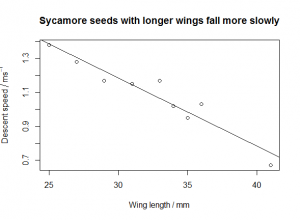nadh.absorbance<-read.csv( "H:/R/nadh_absorbance.csv" )
plot(
A340 ~ Conc.uM,
ylab = expression(A),
main = "Absorbance at 340 nm shows shows linear\nBeer-Lambert law for NADH"
)
summary( nadh.absorbance.model )
Call:
lm(formula = A340 ~ Conc.uM, data = nadh.absorbance)
Residuals:
Min         1Q     Median         3Q       Max
-0.0043482 -0.0020392 -0.0004086  0.0020603 0.0057544
Coefficients:
Estimate Std. Error t value Pr(>|t|)
(Intercept) 2.973e-03  8.969e-04   3.314  0.00203 **
Conc.uM     6.267e-03  3.812e-05 164.378  < 2e-16 ***
---
Signif. codes:
0 '***' 0.001 '**' 0.01 '*' 0.05 '.' 0.1 ' ' 1
Residual standard error: 0.002783 on 38 degrees of freedom
Multiple R-squared: 0.9986,    Adjusted R-squared:  0.9986
F-statistic: 2.702e+04 on 1 and 38 DF,  p-value: < 2.2e-16The slope is 6.267×10−3 µM, which means ϵ is 6.267×103 M. This is very significantly different from zero; however, so too is the intercept, which – theoretically – should be zero. You’ll also note that the Q-Q plot:

qqnorm( residuals( nadh.absorbance.model ) )Is very clearly not a straight line, indicating that the variance in the residuals is not a constant.

1. Species-area requires a log/log transformation$\log{S} = \log{C}+z\log{A}$
logS<-log(c(     9,    5,   3,   2,   2 ))
logA<-log(c( 116.3, 63.5, 7.9, 5.2, 1.3 ))
species.area<-data.frame(logS=logS,logA=logA)
plot(
logS ~ logA,
data = species.area,
xlab = expression("ln( Area of island"/ km^2 *" )"),
ylab = "ln( Number of species )",
main = "Species supported by islands of different areas"
)
species.area.model<-lm( logS ~ logA, data=species.area )
abline( species.area.model )
summary( species.area.model )
Call:
lm(formula = logS ~ logA, data = species.area)

Residuals:
1        2        3        4        5
0.22137 -0.16716  0.00828 -0.25948  0.19699

Coefficients:

Estimate Std. Error t value Pr(>|t|)
(Intercept) 0.40977    0.20443   2.004   0.139
logA        0.32927    0.06674   4.934   0.016 *
---
Signif. codes:
0 '***' 0.001 '**' 0.01 '*' 0.05 '.' 0.1 ' ' 1

Residual standard error: 0.2471 on 3 degrees of freedom
Multiple R-squared: 0.8903,    Adjusted R-squared:  0.8537
F-statistic: 24.34 on 1 and 3 DF,  p-value: 0.01597

log C is the (Intercept), 0.4098 (so C itself is e0.4098 = 1.5), and z is the slope associated with logA, 0.329 km−2. The residual plots are a little difficult to interpret as the sample size is small; and you’ll note the large error in the estimate of log C, which is not significantly different from 0 (i.e. C may well be 1). I wouldn’t want to bet much money on the estimates of C or of z here.

## Statistical testing

If you want a random yes/no answer to a question, like “who should kick-off this football match?” it’s very common to entrust the decision to the flip of a coin, on the assumption that the coin doesn’t care which side gets the advantage.

But what if that trust is misplaced? What if the coin gives the answer “tails – your opponent kicks-off” more often than “heads – you kick-off”, and so gives the advantage to your opponent more often than to you? And, most importantly, how could you tell if the coin is biased before you entrust your decision-making to it?

One answer is to perform a statistical test on a sample of coin-flips, and to see whether the number of heads is lower than you would expect if the coin were fair. In this post, we’ll see how to do this from scratch for coin-flipping, so that when you do more complex tests, you have a better idea of what they’re doing under the hood.

A statistical test is a way of choosing whether to reject a hypothesis. So, before you consider a statistical test, you should have in mind at least one clear hypothesis:

• The null hypothesis, H0, which usually states something like “there is no difference between A and B”, e.g. “this coin has the same probability of coming up heads as tails”.

You might also have in mind another hypothesis…

• The alternative hypothesis, H1, which states something at odds with the null hypothesis: “there is a difference between A and B”, e.g. “this coin is biased, and produces – on average – more heads than tails when flipped”.

However, proper handling of alternative hypotheses will have to wait for another post.

If you collected just a single piece of data – a single coin-flip – then you would have absolutely no idea of whether the coin is biased, because the chance of a single head is exactly the same as the chance of a single tail: 50%, or 0.5. We can plot these two probabilities against the number of heads to produce a probability distribution (technically, a probability mass function) like this: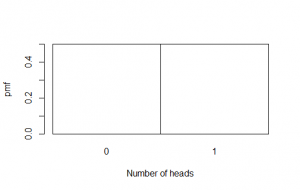The probability of getting zero heads from just one flip of a fair coin, is 0.5; and the probability of getting one head from one flip is also 0.5.

OK, so how about a few more flips? If you did three coin-flips and got not a single head, you might suspect something were awry, but most people wouldn’t bet money on it. Why? Well, if the coin is fair, the probability of getting a head on any given flip is 0.5, and so too is the probability of getting a tail. Furthermore, every flip of the coin is independent of the last – unless you’re a fraudulent coin-flipping genius with terrifying motor control. So, assuming that the coin is fair, and that you are fairly clumsy, the chances of zero heads from three coin-flips is 0.5 (for the first tail) times 0.5 (for the second) times 0.5 (for the third) = 0.53 = 0.125 = 12.5%, i.e. it’s something that will happen more than 10% of the time.

However, if you did thirty coin-flips and got not a single head, you’d be almost certain the coin were biased, and the maths backs this up: 0.530 is, as near as damn-it, a 1 in a billion chance. It’s not impossible that the coin is unbiased – experiments can never rule anything out completely! – but this would be a particularly unlikely fluke.

But what if you got 10 heads in 30 coin-flips? Or 3 heads in 10? Or 8 heads in 40? What number of failures to get a head in a sample of flips would be enough to convince you the coin were biased? Indeed, how many times should you flip the coin to check its fairness anyway?

Let’s take the example of two flips of an unbiased coin.  The possible results from two flips are:

1. HH
2. HT
3. TH
4. TT

The probability of zero heads is 0.5×0.5 = 0.25.

The probability of one head and one tail is 2×0.5×0.5 = 0.5, because there are two different combinations in which you can get a single head and a single tail (HT or TH).

The probability of two heads is 0.5×0.5 = 0.25.

If we plot these probabilities against the number of heads, as before, we get this distribution:

Note that the heights of the bars are in the ratio 1:2:1, showing the 1 way you can get zero heads, the 2 ways you can get a head and a tail, and the 1 way you can get two heads.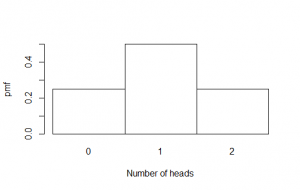For three flips, there are eight possibilities:

1. HHH
2. HHT
3. HTH
4. THH
5. HTT
6. THT
7. TTH
8. TTT

The probability of zero heads is 0.5×0.5×0.5 = 0.53 = 0.125. The probability of one head and two tails is 3×0.5×0.5×0.5 = 0.375, because there are three different combinations of one head and two tails. The same is true of two heads and one tail; and the probability of three heads (zero tails) is the same as for three tails, 0.125. Here’s the graph for three flips of a fair coin; again note the ratio: this time it’s 1:3:3:1.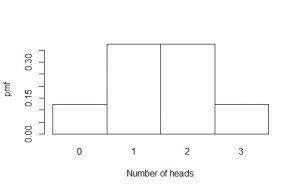And finally, for four flips, there are 16 possible outcomes, each with a probability of 0.54=0.0625:

1. HHHH
2. HHHT
3. HHTH
4. HTHH
5. THHH
6. HHTT
7. TTHH
8. HTTH
9. THHT
10. THTH
11. HTHT
12. TTTH
13. TTHT
14. THTT
15. HTTT
16. TTTT

There is 1 way to get zero heads, 4 ways to get one head, 6 ways to get two heads, 4 ways to get three heads, and 1 way to get four heads.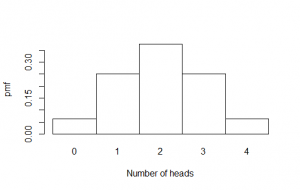You may recognise the ratio of the bars in these graphs from Pascal’s triangle, where each number is formed by summing together the two numbers diagonally above it:

The probability distributions graphed above are three specific examples of the binomial distribution, which can be calculated in the general case using the following formula:$pmf(k, n, P) = \binom{n}{k} P^k (1-P)^{n-k} = \frac{n!}{k!(n-k)!} P^k (1-P)^{n-k}$
• pmf(k, n, P) is the probability of getting k heads in a particular sample of size n, where the probability of a head on any particular flip, P, is 0.5.
• P is the long-run probability of the coin giving you a head, i.e. the ratio of the number of heads to the total number of coin-flips for some extremely large sample. For a fair coin, this will be 0.5.
• n is the number of coin-flips you have sampled.
• (n,k) is the binomial coefficient from Pascal’s triangle, which can be calculated from scratch using factorials (3! = 3×2×1, etc.) if you are a masochist.

For a fair coin, p=0.5, and this formula simplifies to:$pmf(k, n) = \binom{n}{k} 0.5^n$

To see whether a coin is fair, we would like to know how likely it is that the number of heads we get in a sample is consistent with a coin that gives heads 50% of the time and tails 50% of the time. We are much more likely to be convinced that getting 10 heads in 30 flips (33% heads) means the coin is biased, than we would be by getting 0 heads in 1 flip, even though that’s an superficially much more damning 0% heads. So it is clear that our opinion about the coin’s fairness will depend critically on n, the number of coin-flips we collect. This is called the sample size.

In this sort of statistical testing, the first thing we do is to define a null hypothesis. In this case, our null hypothesis is that the coin is fair. Under the null hypothesis, the probability of getting a head on a particular flip is 0.5.

We then assume that the null hypothesis really is true, and we ask ourselves: what probability distribution of results would we get, assuming that the null hypothesis is true? For the coin-flip, we’d get a binomial distribution of results: the number of heads in a sample of size n would be modelled by the equation shown above.

We then collect our sample of coin-flips. Let’s say we collect 30 in this case, so n = 30. We can then generate the precise probability distribution for a fair coin, flipped 30 times. It looks like this: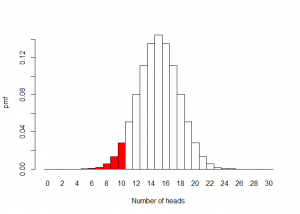Code for (nearly) this graph is:

P<-0.5
n<-30
k<-c(0:n)
pmf<-dbinom(k, size=n, prob=P)
names(pmf)<-k
barplot( pmf, space=0, xlab="Number of heads", ylab="pmf" )
dbinom
The function dbinom(k, size=n, prob=P) is R’s implementation of the probability mass function pmf( k, n, P ) discussed above. The ‘d’ stands for density, because for continuous distributions, the equivalent of a probability mass function is called a probability density function.dbinom(k, size=n, prob=P) gives you the probability of k heads in a sample of size n with a coin producing heads at prob P.

dbinom(10, size=30, prob=0.5) gives the probability of getting 10 heads in 30 flips of a fair coin.

Other distributions have similar density functions available: dnorm (normal), dpois (Poisson), dt (t), df (F), etc.

Here we pass dbinom a vector of values of k between 0 and n, which returns a vector containing the whole probability distribution. The names function can be used to give names to the items in the probability distribution, which is useful for labelling the x-axis of a barchart.

Let’s say that only 10 of the 30 flips were heads. As you can see from the graph above, this is far into the left tail of the probability distribution. The vast majority of possible outcomes of 30 coin-flips contain more than 10 heads. It is therefore pretty unlikely that 10 heads in 30 flips is consistent with the null hypothesis that the coin is unbiased. We would probably be happy to reject the null hypothesis, but can we be a little more objective (or at least consistently subjective!) about the criterion for rejection?Yes, we can. We can work out from the model exactly how likely is it that 30 coin-flips would produce 10 or fewer heads. It’s important that we include ‘or fewer’, because if we’re convinced of bias by 10 heads out of 30, we’d be even more convinced by 5 out of 30, or 0 out of 30. This can be worked out from the cumulative distribution function for the binomial distribution. This is found for 10 heads by simply summing up the the probabilities for 10, 9, 8 … 2, 1, or 0 heads; and more generally by summing up the probabilities “below” a given number of heads. Here is the cumulative distribution for 30 coin-flips:0.04937 is just slightly less than 0.05, or 5%, or 1-in-20.R helpfully has a function for calculating the cumulative distribution function.

pbinom
The function pbinom(k, size=n, prob=P) is R’s implementation of the cumulative distribution function discussed above. The ‘p’ stands for p-value.pbinom(k, size=n, prob=P) gives you the probability of getting k or fewer heads in a sample of size n with a coin producing heads at prob P

pbinom(10, size=30, prob=0.5) gives the probability of getting 10 heads or fewer in 30 flips of a fair coin, which is 0.04937.

Other distributions have similar p-value functions available: pnorm (normal), ppois (Poisson), pt (t), pf (F), etc.

Assuming our coin is fair, there is only a 1-in-20 chance that we would get 10 or fewer heads in a sample of 30. We call this probability the p value. On both graphs, I’ve marked the region where the p value is less than 0.05 in red. Note that the p value is not the same thing as the probability of the coin producing a head, which I’ve symbolised as P above.

qbinom
The function qbinom(p, size=n, prob=P) is R’s implementation of the inverse cumulative distribution function, which was useful for working out which columns should be red on the graph. The ‘q’ stands for quantile. qbinom(p, size=n, prob=P) gives the number of heads in a sample of size n with a coin producing heads at prob P for which the cumulative distribution function first exceeds p.

qbinom(0.05, size=30, prob=0.5) gives the number of heads in 30 flips of a fair coin for which p first exceeds 0.05, so this returns 11 (remember that 10 heads out of 30 has a p value of just under 0.05, so we’d need 11 for a p value of at least 0.05).

Other distributions have similar quantile functions available: qnorm (normal), qpois (Poisson), qt (t), qf (F), etc.

In scientific writing, the results of statistical tests with p values less than 0.05 are called statistically significant, and those with p values greater than 0.05 statistically insignificant. This cut-off at 0.05 is essentially arbitrary, and sometimes 0.01 or 0.001 are used instead, but 0.05 is used fairly widely in science as the threshold for “significant” data.The concept of p-values and the use of statistical significance as a way of analysing data were first proposed  by Ronald Fisher. Fisher’s “significance testing” approach tends to get confused (even by scientists!) with the conceptually different “hypothesis testing” approach of Jerzy Neyman and Egon Pearson, which we’ll visit in a later post. It’s important to know what a p value is to at least give you a chance of avoiding this confusion yourself.The p value is the probability of obtaining a test statistic at least as extreme as the one that was actually observed, assuming that the null hypothesis is true.It is not the probability that the null hypothesis is true, or that the alternative hypothesis is false. It is not the probability the results are a fluke. It is not even the probability that you have rejected the null hypothesis incorrectly. All the p value tells you for this experiment is how probable it is that 10 or fewer heads would be observed from 30 flips of a fair coin: the answer is “not very”.To sum up:

• The null hypothesis was that the coin was fair, so we can therefore assume  that the probability of any one flip coming up heads is 0.5.
• The test statistic we have obtained from our data is simply the number of heads, which is 10. In other kinds of statistical test, the test statistic will usually be something more complex.
• The probability of obtaining 10 heads was worked out using a reference distribution, the binomial.
• Generating this reference distribution requires one additional parameter, and that is the sample size, n, which was 30.
• If we follow this procedure, we get a p value less than 0.05, and therefore we can – perhaps! – say that the data we have collected are inconsistent with the null hypothesis of a fair coin.

All this rigmarole sounds like a lot of effort, and it is. This is why you’re using R. The whole of the analysis above can be done automatically for you by simply typing:

binom.test( 10, 30, p=0.5, alternative="less" )

Exact binomial test data: 10 and 30 number of successes = 10, number of trials = 30, p-value = 0.04937
alternative hypothesis: true probability of success is less than 0.5
95 percent confidence interval: 0.0000000 0.4994387
sample estimates: probability of success 0.3333333


This carries out a binomial test for 10 ‘successes’ (heads) in 30 ‘trials’ (coin-flips), assuming that the coin is fair. The p argument in the call to the function is the probability of success in a single trial, which we called P above, not the p value. The letter “p” tends to get rather overloaded with conflicting meanings in statistics, so be careful about what is is being used to symbolise in any given circumstance. We also specify that the we’re only interested in whether there are less (fewer!) successes than we’d expect. You’ll note the resulting p value is as we calculated above. The test described above is a one tailed test, as we have only checked to see whether the coin is biased towards tails. In general, we’d use a two tailed test, because a coin that produces an excess of heads is just as biased as one that produces an excess of tails, and – without good reason to suspect bias in one direction or the other – we should be prepared for either form of bias. This changes the analysis a little, as we’d be looking at the tails on both sides of the probability distribution, but the principles are the same.

Other kinds of statistical test, such as F, and t, and χ² are more complex, and – unlike this exact test – they only estimate p values based on some additional assumptions, rather than calculate them exactly from first principles. One reason for this is that – for example – calculating precise binomial p values for large values of n requires creating some pretty enormous numbers (because of all those factorials: 30! = 265252859812191058636308480000000). This becomes impractically slow and/or inaccurate, making it more practical to use approximations that are less computationally intractable. However, inexact tests are still doing similar things under the hood. They compare a test statistic (e.g.t) derived from your data, to a reference distribution (e.g. Student’s t) that you’d expect to get assuming the null hypothesis is true (“the means of two samples are the same”), and accounting for any important parameters of the data (degrees of freedom). They then return a p value that tells you how probable it is that a test statistic at least as extreme as the one you actually got would have been observed, assuming the null hypothesis is true.A very reasonable question to ask at the end of all this is: how many times should you flip the coin to see if it is biased? That very reasonable question unfortunately has a very long answer, and will require another post!

# Exercises

1. But what if you got 3 heads in 10?
2. Or 8 heads in 40?
3. And what if you didn’t care whether the coin was biased to heads or to tails, for 8 heads in 40?
4. What is the largest number of heads you would consider significant at p=0.05, for a sample size of 100 flips? You’ll need the qbinom function, which will return the smallest value of k for which the cumulative distribution function is larger than your p value.
5. Produce a barchart of the probability distribution for n=40, like the one shown in the post for n=30. For extra happiness, colour the left and right p=0.025 tails red. You’ll need the col to the barplot function, which can take a vector of colours such as "red" and "white" as an value.
6. Produce a barchart of the cumulative distribution for n=40, like the ones shown in the post for n=30. For extra happiness, colour the left and right p=0.025 tails red. You’ll need the pbinom function, which will return the cumulative distribution function for each value of k.

1. 3 in 10, one-tailed: not significantly less that what you’d expect, given the null.
binom.test( 3, 10, p=0.5, alternative="less" )
        Exact binomial test
data:  3 and 10
number of successes = 3, number of trials = 10, p-value = 0.1719
alternative hypothesis: true probability of success is less than 0.5
95 percent confidence interval:
0.0000000 0.6066242
sample estimates:
probability of success
0.3
1. 8 in 40 one-tailed, significantly less than you’d expect, given the null.
binom.test( 8, 40, p=0.5, alternative="less" )
        Exact binomial test
data:  8 and 40
number of successes = 8, number of trials = 40, p-value = 9.108e-05
alternative hypothesis: true probability of success is less than 0.5
95 percent confidence interval:
0.0000000 0.3320277
sample estimates:
probability of success
0.2
1. 8 in 40 two-tailed, [still] significantly different from what you’d expect, given the null. Note that the p value is double the p value from the previous answer: this is because the 5% is now divided half-and-half between the ‘extremely few heads’ tail of the probability distribution, and the ‘extremely many heads’ tail. This makes it harder for a two-tailed test to give you a significant p value than a one-tailed test. Consequently – as we scientists are a conservative lot – we generally use the two-tailed version of tests unless there is some extremely good reason not to.
binom.test( 8, 40, p=0.5 )
        Exact binomial test
data:  8 and 40
number of successes = 8, number of trials = 40, p-value = 0.0001822
alternative hypothesis: true probability of success is not equal to 0.5
95 percent confidence interval:
0.09052241 0.35647802
sample estimates:
probability of success
0.2
1. The largest number of heads in a sample of 40 that would still give p=0.05 is either 14 (for a one-tailed test) or 13 (for a two-tailed test). Note that the qbinom function returns the smallest value of k such that pmf(k, n, P) p, i.e. it returns the next value of k ‘up’ from the number of heads we’d consider suspicious.
qbinom(0.05, size=40, prob=0.5)
15
qbinom(0.05/2, size=40, prob=0.5)
14
1. Graph of 40-flip PMF. We set up a vector called colorcode containing 41 copies of the word “white”, and then modify this to “red” for the items in colorcode whose corresponding values of k have a CDF of less than 0.025. Note the use of a conditional in the subscript call to colorcode, and that because we want both tails, we need those values with CDFs less than 0.025 (or greater than 0.975).
P<-0.5
n<-40
k<-c(0:n)
p.crit<-0.05/2

pmf<-dbinom(k, size=n, prob=P)
names(pmf)<-k

# Values of k below k.crit have a cumulative probability of less than p.crit
k.crit<-qbinom(p.crit, size=n, prob=P)

colorcode<-c( rep("white", n+1) )
colorcode[ k < k.crit ]<-"red"
colorcode[ k > n-k.crit ]<-"red"

barplot( pmf, space=0, xlab="Number of heads", ylab="pmf", col=colorcode )1. Graph of 40-flip CDF. Note that the p-value for 13-or-fewer plus 27-or-more is actually a fair bit less than 0.05 (it’s about 0.038), but because the distribution is symmetrical, if we took the next two values (14 and 26) and added them to the tails too, we’d get a p value of 0.081, which is larger than 0.05. As the distribution is discrete, we can’t do anything but err on the side of caution and exclude 14 and 26 from the tails.
P<-0.5
n<-40
k<-c(0:n)
p.crit<-0.05/2

cdf<-pbinom(k, size=n, prob=P)
names(cdf)<-as.character(k)

k.crit<-qbinom(p.crit, size=n, prob=P)

colorcode<-c( rep("white", n+1) )
colorcode[ k < k.crit ]<-"red"
colorcode[ k > n-k.crit ]<-"red"

barplot( cdf, space=0, xlab="Number of heads", ylab="cdf", col=colorcode )
segments( 0, p.crit, n+1, p.crit, col="red" )
segments( 0, 1-p.crit, n+1, 1-p.crit, col="red" )